A Viscosity Hypothesis and#8211; That the Presence or Absence of Viscosity Separates Relativistic and Quantum Systems Based on the Simplest Possible Theory of Everything
Journal of Physical Mathematics

# A Viscosity Hypothesis – That the Presence or Absence of Viscosity Separates Relativistic and Quantum Systems Based on the Simplest Possible Theory of Everything

Lawrence M*

Maldwyn Centre for Theoretical Physics, Cranfield Park, Burstall, Suffolk, UK

*Corresponding Author:
Lawrence M
Maldwyn Centre for Theoretical Physics
Cranfield Park, Burstall, Suffolk, UK
Tel: 595982298
E-mail: [email protected]

Received Date: August 03, 2016; Accepted Date: September 27, 2016; Published Date: September 30, 2016

Citation: Lawrence M (2016) A Viscosity Hypothesis – That the Presence or Absence of Viscosity Separates Relativistic and Quantum Systems Based on the Simplest Possible Theory of Everything. J Phys Math 7: 200. doi: 10.4172/2090-0902.1000200

Copyright: © 2016 Lawrence M. This is an open-access article distributed under the terms of the Creative Commons Attribution License, which permits unrestricted use, distribution, and reproduction in any medium, provided the original author and source are credited.

Visit for more related articles at Journal of Physical Mathematics

#### Abstract

A simple framework for our universe in which the basic constituents act as a background upon which actions by composite particles, composed of those same constituents in motion, act and whose presence or absence from volumes give rise respectively to relativistic and quantum systems. Where the background exists, all composite particles experience energy loss in motion due to viscosity and a maximum velocity and where the background does not exist, there is no energy loss in motion and no maximum velocity. The framework is based on the simple premises of the one size of fundamental building block, the meon, two types of energy, one composite loop form of particle and only three dimensions. Composite loops formed from the unit meon building blocks during different inflation events produce different sizes of fermions, nucleons and atoms, but produce a type of universe with symmetries similar to ours as the inevitable outcome of a successful inflation event. The rate of expansion after a big bang is a function of the size of the electron formed during inflation and that size defines whether the expansion will eventually succeed or fail.

#### Keywords

Universe, quantum mechanics; Inflation; Expansion; Black hole; Symmetry; Loops; Stacks; Chain star; Meon; Lepton; Anomalous magnetic moment; Quark; Electron; Viscosity; Dark Matter; Theory of Everything; Dark energy; Arrow of time; Second law of thermodynamics; Viscosity redshift; Prequark; Steady state

#### Introduction

Due to size constraints the abstract above was kept short and the following is a more detailed account of what this paper will cover:

It is shown that starting from a very simple set of premises it is possible to construct a framework with properties significantly similar to those of our currently observed universe in which the basic constituents act as a background upon which actions by composite particles, composed of those same constituents in a different dynamic state, act and whose presence or absence from specific volumes give rise respectively to relativistic and quantum systems. The conclusion is that where the background, as described, exists then all composite particles experience energy loss in motion and a maximum velocity and where the background does not exist, there is no energy loss in motion and no maximum velocity.

The background consists of adjusted-Planck volume zero mass black holes which are each comprised of a pair of particle and antiparticle (named meon and anti-meon respectively) whose motions when wholly or partially merged are rotational, vibratory and translational. When unmerged, requiring the same amount of energy every time, the result is a pair of particle and anti-particle chasing each other with one-sixth opposite sign electron charge each. The pair can chase other pairs to form composite loops. Three-pair loops are our normal matter with other size loops, plus specific non-symmetric three-pair loops, being dark matter.

The foundation is based on the simple premises of the one size of fundamental building block, the meon, two types of energy, one composite form of particle and only three dimensions. The loops of three-pairs are the leptons and quarks. Shear viscosity is the reason for a maximum speed through the background medium when particles travel through it, for the arrow of time where all motion loses unrecoverable energy and for an additional redshift of photons. That photons travel at a terminal velocity against the local background defined as the local speed of light shows that there is internal chasing between meon and anti-meon. Where no background is present, the result is non-locality with motion above c and a quantum mechanical environment.

The motion of the meons and anti-meons around the loops can be split into the balancing of mass energies and the resultant motion of charge energies. For every mass energy that a meon or composite particle has, it will always have a similar amount of charge energy, and the same, but opposite, for each meon, anti-meon or anti-particle energy. An analysis of dimensionality, deeper than mass, length and time, shows that the product of volume and shear viscosity is constant, so where all meons have the same volume they are all affected equally by the background shear viscosity and composites will lose rotational rate proportional to distance travelled almost regardless of loop frequency.

The nature of what is currently described as energy is shown to be both a counting phenomenon and a vector property. How what is described as the energy for the big bang was generated and where it is being used currently is shown to affect our understanding of time, mass, light speed, normal and dark matter and the background within which matter exists.

The overall movement of what is currently described as energy starts from the background state of the universe, goes through the formation of loops and gradually returns to the background through the action of the viscosity of the background. It is shown that there can be only one underlying set of the laws of physics and these laws will be the same everywhere and fail nowhere. Composite loops formed from the same meon building blocks during different inflation events can produce different sizes of fermions, nucleons and atoms, but a type of universe with symmetries similar to ours is the inevitable outcome of a successful inflation event. The presence of observable failed inflation events randomly within the background allows big bang and a form of steady state system to coexist, with earlier failed inflation events forming seeds for later gravitational accumulation during our successful big bang. The rate of expansion from a big bang is a function of the size of the electron formed during inflation and that size defines whether the expansion will eventually succeed or fail. It is also explained why only positive masses are observed, and why some particle configurations and orbits are stable.

In the framework it is shown that key paradoxes are not paradoxes, with both interpretations correct given a deeper understanding. The ideas proposed here may be viewed as speculative because they start from a prequark framework. This physics beyond the standard model derives its strength from the number of aspects of the universe that it explains, including what energy really is, how mass arises, what inertia is, why particles have spin in units of ½ h, why electrons have 720o of rotation, why all electrons have the same charge size, as do nucleon stacks, why particles have internal magnetic moments, why the second law of thermodynamics exists, why there is an arrow of time, why there is a maximum speed for particles, why stable states exist, why tired light may reduce the need for dark energy or the size of the universe, why there is no matter/anti-matter imbalance, what dark matter is likely to be, what is likely to be the physical reality underlying zero point energy, why physics is the same everywhere and breaks down nowhere and why we have only one universe with threefold symmetry within nucleons in matter.

This paper is not written from an arrogant perspective. The author does not suggest that everything in the paper is unquestionable, only that the current interpretations are open to reinterpretation and that what is included here is one possible solution which happens to solve many paradoxes and provides answers to many otherwise unexplainable observations. It does not doubt one single mathematically accepted observation, although it provides reasons to interpret some of them differently.

The relativistic and quantum realms are understood from fundamental differences  to be inconsistent. Even discounting the different treatments of time, size and position, the wave versus particle treatments are difficult to explain . Non-locality has been shown  and yet nothing is supposed to exceed local light speed .

The solution to the many paradoxes between relativity and quantum mechanics starts by understanding that there are two entirely different environments within the universe – one where a ‘background’ of basic merged fundamental particle and anti-particles exist against which motion of composites takes place, and one where the background is excluded. This paper will show how and why this happens and how the motion of the photon is effectively a proof of this difference and of the existence at the most basic level of negative fundamental mass, although not of negative mass of composite particles or with the properties usually ascribed to it.

The quest to explain everything has progressed in fits and starts. Each new addition to understanding has been placed within the existing jigsaw of knowledge, either replacing a piece seen no longer to fit or adding to the whole which needs to be solved.

Unfortunately it appears that we have been using the jigsaw pieces to try to solve the wrong picture. They may fit together in places, but do not show the correct picture overall. History has permanently nailed so many pieces in the wrong place that it is difficult for any other picture to be contemplated as a replacement.

What this paper sets out to do is to show what the replacement picture should look like. It shows that many of the pieces are perfectly valid, but just stuck in the wrong place. And it shows that as we delve deeper into nature the mathematics needed get simpler.

As will be explained, what we describe as ‘mass’ is the frequency of rotation of the loop composites, which is always measured as positive, and all loop composites always have a balance of internal energies and seek to balance their external energies to total zero overall. This is why some configurations of composites in atoms or orbits are stable and shows why we need to reinterpret what we mean by energy as well as inertia.

The fundamental particle pairs that, as will be shown, comprise photons, are affected by the shear viscosity of the background universe equally, almost regardless of the photon frequency. This viscosity also provides a maximum speed of travel for all particles, which we call light speed c. The same viscosity affects the fundamental particle pairs that comprise non-photon composites, providing an arrow of time as they lose rotational rate. Where there is no background, photons are not affected by viscosity and non-locality exists, along with all quantum mechanical effects.

The paper connects the very large to the very small and provides a logical and the simplest possible foundation for a theory of everything.

#### Significance and Objectives

The paper sets out to show where relativistic and quantum mechanical systems can exist. At the smallest end of the scale is a simple foundation out of which can be made the particles that we can observe and the basis for those that we cannot. Based on those most simple foundations automatically appear the symmetries we observe and the explanation of why we can only observe a fraction of what exists in the universe. The significance of the framework proposed can be seen in just two different scale examples explained later –contributions to magnetic moments in the charged leptons and how and why failed inflation events occur and what they look like afterwards to an observer.

Based on the simple foundations proposed, as published previously , and as corrected with the missing tables , the relationships between properties, such as mass, velocity and energy, and the dynamics of the foundation particles, the underlying laws of physics in any part of the universe are simple and cannot be any different. However, the actual sizes of properties are the result of the local inflation event in which the loop composites are formed. In this framework there can be no singularities since the building blocks are the densest particles possible.

The significance of this paper is that it solves many conflicting issues across physics from the very small to the very large, using only very simple foundations. It also shows how viscosity separates the relativistic and quantum environments.

Basics

The framework used in the foundation equations here is based on the Triple-adjusted Planck Units (TAPU) set of units and property values established in the foundation paper  and as corrected .

The main relationships and principles will be outlined briefly. All the equations here use only Planck values, unless specifically mentioned otherwise. The Planck, or adjusted-Planck, values are call ‘maximal’ in that they represent either the largest (eg velocity, c) or smallest (eg distance, LP) that is possible for that property.

The analysis is in three stages, where Xx represents any property, such as mass, length, time etc:

a) Eliminating 2π for simplicity from the generally accepted Planck equations denoted Xp to give Adjusted Planck Units (APU), denoted Xo

b) Eliminating G to give Double-Adjusted Planck Units (DAPU), denoted X*

c) Eliminating h to give the final Triple-Adjusted Planck Units (TAPU), denoted XT

The most basic two formulae for defining a Planck unit sized system are the gravitational force equation F =G M2 / L2 =Q2 c2 / L2 and the quantum angular momentum equation h=M C L. The normal usage of the latter is to define a Planck mass Mp and Planck Length Lp such that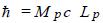and /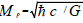. Unfortunately this introduces the 2π factor in many equations, where it serves only to confuse.

The preferred definition, to be used here as a starting point, is to define the system without the 2π factor, which is the first adjustment. This adjustment is split equally between the mass and length units.

Initially the APU mass Mo and APU length Lo are related by h=Mo c Lo and is defined to be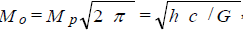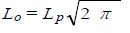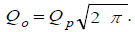In the second stage, however, to achieve the right relationship between M and L in property space, as described below, requires looking at the force equation at the same time.

Rearranging to give F L2 = G M2 = (Q c )2 provides the simple relationship that the APU mass Mo and APU charge Qo are related such that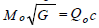. Since the latter equation does not include Lo it is not immediately apparent that compared with the Planck properties Mp and Lp there is a need to adjust both by the factor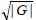in addition to the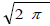factor, so that now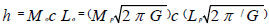if the latter factors are distributed in the same way as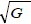.

It is possible now to define the second adjustment such that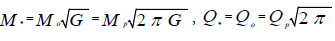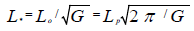with h=M*c*L*, the basic DAPU units, where Q* is the DAPU charge. This is the maximum charge based on symmetry with the maximum mass and is not the electron charge, which is considered later.

The result is the foundation of a DAPU property set and units based on

h=M*c*L*

and

F*L2* =M2*=Q2 c2 = h c

which was the objective of the second adjustment, in that the formulae exclude G. The dimensionality of G will be shown to be zero later.

This is the most basic set of Planck properties that can be devised using only two universal constants h and c. However, as shown in the third adjustment stage, this is not the minimum number of constants required to establish relationships between the properties.

The relationship between M* and Q* is simply M*=Q*c with the deeper relationships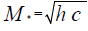and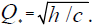.

The subsuming of G within the mass and distance units eliminates the difference between gravitational and inertial masses, since there is no longer any purely gravitational mass.

The subsuming of G within the APU mass Mo to produce the DAPU mass M*, and the APU length Lo to produce the DAPU length L* would seem to ignore the units of G, effectively treating G as being without units. This is not the case since G has units of m3kg-1 S-2, but it is necessary to show that, based on Planck sizes, these units cancel completely to leave only a ratio.

A consideration of the standard laws of nature and the fundamental constants through a form of dimensional analysis shows that if each property at its maximal Planck size is assigned an appropriate dimensionality, every fundamental constant, other than c, will have a total dimensionality of zero, or to state the reverse – every property that has dimensionality of zero is a fundamental constant.

The dimensionalities of the main SI, NSI, APU, DAPU or TAPU properties in terms of a hypothetical dimension Y that emerge from the consideration are: M*=Y+1

Mass M*=Y+1 Velocity c=Y+2

Length L*=Y-3 Energy E*=Y+5

Charge Q*= Y-1, Time T*=Y-5, h=Y0 G=Y0

The units of G are m3kg-1 S-2=Y-9Y-1 Y+10=Y0 dimensionality and h has units of m2kg S-1=Y-6Y+1 Y+5 dimensionality. So the units of both h and G are actually irrelevant because they represent fundamental constants with zero dimensionality. Similarly Boltzmann’s constant has units of J K-1=Y5Y-5=Y0 dimensionality as well.

Thus adjusting the APU mass to the DAPU mass, and APU length to DAPU length, involves only multiplying or dividing by the ratio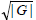as a dimensionless number, and does not affect the dimensionality of the units of mass, charge or length, other than changing the sizes of the base Planck mass, charge and distance units. This stretches the current property space into the more symmetric DAPU property space which does not affect the current property space topology at all and which is different to treating G to be equal to one.

It is now necessary to make the final third adjustment to produce the most simple definitions possible of mass and charge, that is the TAPU definitions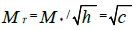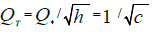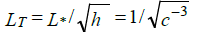and to show their simple relationships to all other properties through a new ratio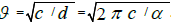.

The base formulae are now: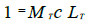and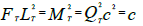The new TAPU sets are based around the XT set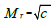and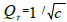.

One new relationship to emerge from the dimensional analysis is that the product of Shear Viscosity η* of dimension Y+9 and Volume V* of dimension Y-9 is equal to h in DAPU, so is a constant. Thus any system where all component fundamental particles, like the meons, have the same volume will experience the same effect due to shear viscosity when travelling in the background environment. As will be shown later, this means that the red shift of a photon is dependent almost entirely on the distance travelled by the individual meons rather than the frequency of the two loops that comprise the photon. Tired light is a distance phenomenon, not frequency dependent except at very high frequencies.

It is also worth noting how the current equation relating energy and time, instead of position and momentum in the original Heisenberg relationship , in APU was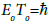and now becomes ETTT=1 in TAPU, and that our original target base has now been reached in terms of manipulating and simplifying formulae for use in explaining the framework in our type of universe.

Our ‘type’ of universe is one with 3-fold symmetry in nucleons, atoms and components, dark matter outweighing normal 3-fold symmetrical matter and three spatial dimensions. These are all inextricably linked in the ‘chain then loop’ proposals described below using only the one type of foundation particle/anti-particle.

Foundations

The foundation is a volume which is composed of a merged particle and its anti-partner. When completely merged, nothing is observable from outside that volume.

The particle, which will be termed a ‘meon’ has positive properties which may be described as fundamental mass and fundamental charge, although these are not necessarily what we understand as normal mass and charge, as will be explained. The anti-partner has negative properties. The meon and anti-meon always appear together as a pair, so the term ‘pair’ will mean exactly that – but only when the two are completely unmerged. When the two are partly merged, they will be termed a zero-mass black hole ‘ZMBH’, of adjusted Planck volume. Figure 1 shows the three states of the meons – ZMBHs, pairs and individual.

The sizes and strength of the actions of mass and charge are equal and are the same for the meons, and anti-meons, as explained in the previous foundation paper  in terms of the dimensionality of physical properties. What the dimensionality section shows is that Planck’s constant h, the gravitational constant G and permeability u− are constants because they have zero dimensionality – they do not depend on any other values. They are actually ratios set by the use of SI units. This paper uses triple-adjusted SI units, adjusted as explained, so that there is no need to include h or G in formulae. However, for clarity h is used occasionally to emphasis what the normal formulae would look like, but its value in these formulae is equal to 1 in new SI units (TAPU). So the strength of a mass field and charge field of equal fractional Planck value is the same.

Myriad ZMBHs are what our universe is composed of, initially and as the ‘background’ within which all relativistic events occur. Where there is no background, as will be explained, there are no relativistic effects. The ZMBHs spin, move, rotate and vibrate to transfer forces – these are the force carriers, not the bosons. Composites, loops made from unmerged pairs, affect the ZMBHs and the ZMBHs affect the composite loops.

There is only one size of ZMBH, which is the triple-adjusted Planck unit (TAPU) size and can be defined simply as size equal to the unit 1 for fundamental mass, fundamental charge and volume. When completely merged, there is an empty volume of 1, with no properties observable. The ZMBH background is both a continuum, overlapping everywhere when completely merged, and yet indivisible in that the unmerged constituents cannot be divided. Only in certain circumstances can there be volumes without a ZMBH background, as will be explained later.

To unmerge a ZMBH into meon and anti-meon takes a specific amount of energy every time. The result is a meon and anti-meon, each spinning about its own internal axis (from now on this motion will be defined as ‘twisting’ to differentiate it from the motion of meons around loops, as explained below, which will be called rotation and the charge-angular momentum of the meons in any loop which is described as the spin of the loop and which is what is usually described as the spin of a fermion). It is proposed as a foundation assumption that the subsequent twisting motion of the meons against the background ZMBHs generates one-sixth the electron charge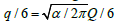, where Q is the TAPU Planck fundamental charge, previously shown as QT, with sign dependent on i) the spiral orientation of twist versus direction of motion and ii) the original meon fundamental charge. The twist mass energy equal and opposite to the charge energy has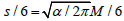where M is the TAPU Planck fundamental mass, previously shown as MT. The motion of the pair is of one chasing the other, as if they were trying to remerge, which will be explained in the section on the hierarchy of zeroes of total energy.

The fundamental charge Q and the one-sixth electron charge q/6 are not necessarily the same property, but are assumed to act in the same way and this is shown to be reasonable in the later analysis of mass and charge currents for loops where half of the circumferential charge current arises from the differential dynamics of meons and antimeons around an electron loop.

In an event where ZMBHs are being unmerged in large quantities to form pairs, which is the start of a big bang, the result of many pairs mixing and chasing each other is the formation of chains. The chains can have any length, but will be broken and reformed many times. The likelihood is that a chain will catch onto its own tail to form a loop. Like the chains, the loops can be any length but the greatest probability will be for the shortest lengths. Assuming the smallest loop to have two pairs, the loops will be 2, 3, 4, 5 and greater number of pairs in length, with the shorter ones more prevalent. In each loop the meon pairs chase, as will be described later in the consideration of the structure of the electron, so that there is always alternating meon and anti-meon along the chain that forms a loop. Figure 2 shows a loop composed of three pairs in motion, chasing each other, at angular frequency w.

Figure 2: Three meon pairs chasing each other in a loop around C at angular frequency w.

When originally unmerged, the pair generates twist charges +q/6 and –q/6, with the sign of charge on each being dependent on the initial direction of travel of the pair, although the only charge combination initially for any pair is +-. Subsequent mixing of pairs will result in only four possible twist charge combinations for any pair ++, +-, -+ and --.

Due to the assumed existence of only one fundamental size of meon with one size of energy necessary to separate out a pair from being a ZMBH, then all composite loops, formed from pairs, will have charges in multiples of units of zero or ±1/3 q. This will be the case for all loops formed in whatever manner, including failed inflation events described later, with the maximum loop charge being the product of the number of pairs in the loop and ±1/3 q or zero.

Loop identities

Taking the loops comprised of three pairs, the 3-loops, the identity of the loops can be defined by the total size of their electronic charge and their internal symmetries – meaning where in the loop the onesixth electron charges sit in relation to each other. The possible charge sizes for 3-loops are 0, 1/3, 2/3 and 3/3q electron charge, each positive or negative apart from the zero charge case.

The motion of the meons and anti-meons in chasing around the loop can be considered as the loop itself rotating about a central axis perpendicular to the plane of rotation of the meons/anti-meons. Loops always rotate.

The loop with zero total electronic charge (neutrino or antineutrino dependent on definition) can be symmetric internally with positive and negative one-sixth charges alternating around the loop, which is actually a symmetric form of hidden 3-fold asymmetry. Asymmetry here refers to pairs in a loop and will be used later more generally to differentiate between symmetric lepton loops and asymmetric quark loops when in stacks. The zero charge loop can also be 2-fold symmetric with three positive one-sixth charges facing three negative one-sixth charges across the loop. The zero charge loops can also be completely asymmetric with no symmetry. As will be explained more later, where loops do not have 3-fold symmetry they are dark matter, unable to interact with 3-fold symmetric loops, or stack, except via gravity and charge. So non-symmetric zero charge loops will not, in usual terminology, ‘feel the weak force’ and it may appear that there are more flavours of neutrino than the expected three – those which can stack when they are 3-fold symmetric.

The 3/3q charge loop can only be perfectly symmetric, with each meon twisting to generate one-sixth charge of the same sign. Again this is a symmetric form of hidden 3-fold asymmetry.

The 1/3q charge loop can only be asymmetric with 2-fold asymmetry or non-symmetric, as is the case for the 2/3q charge loop. It should be obvious that the fractional charge asymmetric 3-loops are what we call quarks and the symmetric loops, including the asymmetric zero charged loops, are what we call leptons, with all eight 3-loop charge permutations being our fermions.

Further stable structures can be made from combining numbers of these 3-loops if the result can be made rotationally symmetric along their axis of rotation. The most obvious form is a stack of loops with all axes of loop rotation coincident and planes of rotation parallel, like a stack of dishes, requiring that their rotational asymmetries be balanced overall to be stable.

This is fine for the symmetric zero and 3/3q charged loops because they can exist separately. However, the asymmetric loops need to be stacked so that their asymmetries cancel each other, otherwise the stack will not be stable. This requires a stack of three 3-loop quarks, a 3-stack, whose asymmetries are at 120 degrees offset to each other when viewed along the mutual axis of rotation of the loops. This is the basis for our three-colour quark colour force framework. The need for symmetry in a stack does not preclude the existence of symmetric loops within a stack. Since the symmetric leptons have hidden 3-fold asymmetry there are good reasons to conclude that a balanced asymmetric 3-stack requires 3-loop symmetric end ‘caps’ to effectively hide the asymmetric loops from the local environment.

Loop masses

What is described as the mass of the loops is derived not from the underlying meons, but from their motion around the loop. Each meon should have energy EM+=(γ-1)Mc2 in the loop, where M is the TAPU Planck fundamental mass (M+ for a positive fundamental mass meon and M- for a negative fundamental mass anti-meon) and γ the relativistic velocity factor for the meon in its motion around the loop. Each anti-meon should have opposite energy EM-=-(γ-1)Mc2. So the total energy due to motion of fundamental mass is zero. This is the case for all energies within the loop where even the one-sixth q twisting charge energy is a balance for the twist mass energy.

These two simple equations are actually too simple, although they show the concept nicely. It is necessary to consider all the energies at work, which includes the twist energy on the mass energy side, so the actual equations are: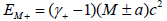and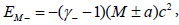, where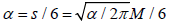and can be either added to or deducted from the fundamental mass energy. The same equations apply to the charges of the meons, giving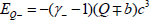and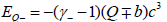where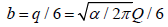.

The γ+ here represents the velocity factor for each meon. These four equations are the main ones as shown later that are needed to help resolve the relative magnetic moments of all loops, plus the circumferential and radial electric fields in motion. As previously mentioned the framework used is that the positive meon has positive fundamental mass and charge, and the negative the reverse.

The total mass energy of a chain would differ depending on its charge. The electron chain would have total q charge and sc2 twist energy, as explained later, and the neutrino zero of each, with quarks fractional in each. But in a loop, each meon or anti-meon needs the same mass angular momentum to remain in a stable loop. So the meons and anti-meons adjust their radii of rotation around the loop so that each has the same size angular momentum, positive or negative. This will be explained more later, but the effect means that each M+ has h+ and each M- has h- , where h± is not necessarily the same as h, in the loop, totalling zero overall. Now the sum of the loop mass energies, through the differential rotational velocities, even though all rotate at frequency w, is zero.

This is equivalent to a deflection of some flat field which might be termed ‘space-time’ except for the quantisation of time into the loops so there is no equivalent background time for ZMBHs. In deflection terms, the depths of all deflections affect each other, with a maximum set by the deflection of a single twisting meon or anti-meon. But for a loop, it is effectively the area of the loop which sets the amount of deflection of space – what we term the ‘mass’ of the loop. The charge of the loop is also a factor as a multiplier but does not change the discussion here.

It is the interaction of one type of energy in one meon or loop with the same type of energy in another meon or loop which underlies their mutual interaction, even though all meons and loops always have a total energy equal to zero. With only fundamental mass and fundamental charge energies, this means there must be deflections of independent flat fields for both charge and mass.

The energy of a loop is also defined by its frequency of rotation wloop such that the simple equation should be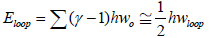Where wo is the Planck angular frequency. However, in view of the fractional a and b energies, this is not quite right. In order for the loop to remain stable, regardless of the mass angular momentum of any meon, as mentioned earlier, the size of each angular momentum should be the same size, positive or negative, so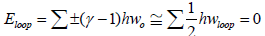As will be shown later for the electron analysis, although each meon has the same angular frequency w, the actual angular momentum of every meon in all loops except the symmetric neutrinos may be either h or h ±=1.0017h. To keep open either possibility, h± will continue to be used in the paper, although it could prove to be that h±=h. So although the mass energies sum to zero overall for all loops, as do the angular momenta, the relationship between energy and angular frequency is not the simple version. This also applies to the mass formula for a meon.

The motional energy of the meons can also be written simply for fundamental mass as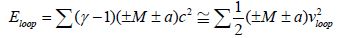Where vloop is the average meon velocity around the loop and is less than c. However, in this instance, the energy depends on how many of the meons have positive or negative a energies and their actual velocities differ depending on whether the a is positive or negative. This will be explained more below.

In the case of a loop with zero overall charge, the a energies sum to zero and the equation becomes the simple version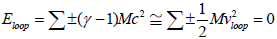So the two methods of describing the meon motional fundamental mass energy are interchangeable - with appropriate care. What we describe as the mass or energy of a loop is just the size of the two balancing positive and negative energies, even though they always sum to zero.

The same equations apply to the meon motional fundamental charge energies, replacing M by Qc, and the energy sizes are identical, adjusted only by c for charge when working in TAPU units. These equations can also be used as the base of the charge current which is one of the components of the magnetic moments of the loops as will be shown below.

The summing of the fundamental M and Qc energies, for every loop, to zero overall means that what we describe as ‘energy’ is really just a counting mechanism relating the frequency of loop rotation to the depth, and direction, of deflection of each mass or charge field. Even relative motion will not make the energy sum anything different to zero overall. However, the maximum deflections by a loop overall will be limited to be same as that of an isolated twisting meon, thus providing a maximum loop frequency equal to the TAPU Planck frequency, with maximum meon relative velocity of c. The actual maximum energy balance depends on how much mass angular momentum any meon has in total.

Loop properties and dynamics

Whilst the sum of the meon motional S(±M±a)c2 fundamental mass and twist energies can be described as the loop mass, the sum of the meon motional (±Q±b)c3 fundamental charge and one-sixth electromagnetic charge energies is the spin energy of the loop – in both cases although they sum to zero, we usually consider only one side of the zero total balloon or balance. If we consider the 3/3 q charged 3-loop, this is the electron and the simple equations imply that the mass energy of the electron is identical to its spin energy where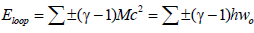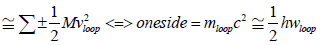These energies are not usually connected in this way because the spin of the electron is taken as 1/2 h, missing out the wloop factor. The more complex equations show that each loop needs to be considered meon by meon, as will be shown for the leptons in Tables 1 and 2, but the relationship between mass and frequency remains with h± angular momentum.

Type +M -M +Q -Q H/S/Any inner/outer H/S/Any inner/outer
T1 +a +a -b -b H + inner S - outer
T2 -a -a +b +b S + outer H - inner
T3 +a -a -b +b H + inner/Any H - inner/Any
T4 -a +a +b -b S + outer/Any S - outer/Any

Table 1: Pair combinations of H and S giving types Tx.

Type e- e+ vs va d- d+ u+ u-
T111 ?
T222   ?
T333     ?
T444     ?
T344       ?
T334       ?
T123       ?
T124       ?
T134         ?
T133         ?
T144         ?
T112         ?
T234           ?
T122           ?
T244           ?
T233           ?
T223             ?
T224             ?
T113               ?
T114               ?

Table 2: Combinations of types Tx to give the fermions.

This identification of the mass of the loop as based on the frequency of rotation of the meons around the loop shows why the property called the ‘fundamental mass’ M of a meon is not the same as the ‘mass’ of a loop. The latter will always be a positive frequency, whereas the former may be positive or negative and may not correspond to the normal interactions expected of masses. The assumption here for meon fundamental mass interactions is that same type of masses attract, opposites chase, as explained later.

What this loop framework shows is that the simple spin of a loop is 1h, and the ±1/2 belongs to the frequency, so the loops have twice the frequency expected at normal energies. This is discussed in the section on the electron below and is why electrons appear to have 720 degrees of rotation, shown by the electron spin g-factor being slightly more than 2 rather than 1.The factor 2 also appears in the section on the electron showing how the charge current arises both from offset rotational radii of meons with their fundamental charges as well as from the six q/6 charges rotating around the loop.

In this explanation the consideration of the motion of both the Q and q/6 charges leads to the non-zero magnetic moment of the charged loops, contributions to the anomalous magnetic moments and an obvious reason in the planar nature of the loops why they can be described as spin up or down and why there cannot be any magnetic monopoles.

Importantly it must be noted that because the mass of any loop is proportional to its rotational frequency rather than the underlying number of meons in the loop, the length of the loop, however many pairs it contains, is independent of the mass of the loop. The same is the case for the spin of the loop, so all single loops (including all fermions) are spin ± 1/2 , in accepted usage.

So a 3-loop of radius x has a mass of y, and a 4-loop of radius x also has a mass of y and both are spin ± 1/2. However, the magnetic moment of non 3-loops will be different because the net current flow, radial and circumferential electric fields will be different. This gives a way of identifying different pair-number dark matter loops from identical charge and mass normal matter loops.

The simple dynamics of meons in a loop is given by

h=Mvloopgloop and vloop=gloop wloop

so the smaller the physical size of the loop, the larger is its energy, but the extra a and b energies make the actual equations more complex. It is always the case though, that v=rw for all meons individually.

Apart from the charge and gravitational formulae, these few equations describe the only laws of physics needed at this fundamental level and will be used in their more complex form to analyse the electron below.

Matter and anti-matter

Currently the basic assumption on matter/anti-matter asymmetry is that somehow there is an excess of matter over anti-matter and after mutual interaction, only the matter excess survives. This is not the case in the framework proposed here.

Consider a chain of pairs of any number travelling across a theoretically-existing flat surface. The chain encounters an obstacle which deflects the chain either right or left so that it catches its own tail. One version will become a clockwise rotating loop and the other an anticlockwise rotating loop. If the clockwise loop, knocked to the right in this thought experiment, is defined to be ‘spin +1/2’ and the anticlockwise as ‘spin -1/2’ it is apparent that the spin energies are the same and the mass energies – the loops’ rotational rates – are also the same. The charges of the loops will also be the same since the meons have not changed twist orientation. How then to define a matter particle or an anti-matter particle?

The underlying difference can only be investigated by switching the time direction for every property of each meon. This means the initial direction of travel of the chain, the twist orientation of each meon and also the underlying identity of each meon which has to become an antimeon and vice versa.

So now the meon twisting right hand screw along one spatial direction (forwards), generating negative one-sixth electron charge, will become an anti-meon also twisting right hand screw along the opposite spatial direction (backwards), generating positive onesixth electron charge. The difference is also that the chain previously deflected right will now form a spin -1/2 loop instead of the earlier spin +1/2 loop since the chain travel direction is reversed and the obstacle is in the way of its new path.

However, in both cases, for both deflections, we could choose the opposite framework in which to view the orientation of spin of the loop. So it cannot be that the definition of matter versus anti-matter can include any spatial orientation.

The only property that provides an unambiguous definition that can be used to define matter and anti-matter is the sign of charge of the loop. If the positive charge is matter, then the negative charge of the electron makes it an anti-matter particle, as would be all other negatively charged loops. Thus all neutral atoms are balances of matter and anti-matter, and all atomic photon emission energies will be identical whether the atoms are composed of neutrons and positively charged protons or anti-neutrons and negatively charged anti-protons with balancing electrons or positrons respectively.

The neutrino could then be defined as both matter and anti-matter since it has no overall charge. Even if a specific position for the start of the loop is defined, so that it would be possible to call one matter and the other anti-matter and rotating either loop by 60 degrees would convert one to the other, this would be the same spatial framework change as for spin and so not usable. The anti-loop of a positive charge spin -1/2 loop is a negative charge spin -1/2 loop. So a photon, as shown below, being loop and anti-loop rotating in the same sense, is a perfectly balanced composite of matter and anti-matter.

Since unmerging ZMBHs produces a balance of fundamental charges as well as of one-sixth electron charges in the twisting meon and anti-meon pair, there can be no matter/anti-matter imbalance in the universe.

Quantum and Gravitational Orbits

The existence of both mass and spin energies in all loops, considering just the mass side of energy interactions, implies that the motion of both energies should be included in the gravitational orbital equations of motion, making them identical to the quantum mechanical versions. This is also seen in the energies within the photon, where both mass and spin energies need to be considered in the relativistic deflection of their motion past the Sun, so although we measure 1 hw of photon energy, the deflection shows that 2 hw are present .

With the new TAPU units, explained in the foundation paper  showing that both G and h are dimensionless ratios, (each property M, Q etc is now in the new units) the new gravitational and quantum orbital formulae at Planck values become: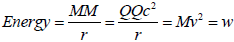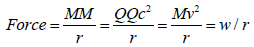However, what is measured in a gravitational orbit is still the kinetic mass energy only, since the kinetic energy of spin can only be measured with spin energy, even though the kinetic energy of spin exists.

The spin energy itself only appears in the equation above when the two bodies have mainly aligned spin components – as is the case for small quantum mechanicals system. For randomly spin-aligned bodies, there will be little overall effect.

In the energy equation above, the kinetic side, since M=QC and there are two different energies in motion, should really be displayed as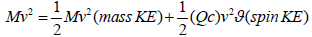where ϑ is a factor for the relative alignment of overall spins in the two bodies, equal to one when both have all loop spins aligned parallel and in the same sense.

This means that the actual total energy of all stable orbits is always zero. That is why the orbits are stable. To move to another stable orbit requires that the energies, potential versus motional, change equally on both sides of the equation. For electron orbitals this is achieved by adding a stack of photon loops of the correct energy onto electron loops or removing them from existing electron-photon stacks and adjusting the orbital size smaller or larger. To be stationary requires the photon double loops of electron and positron to unmerge and stack, probably produced by impact onto the existing stack or single electron loop. Further impact of the appropriate energy may dislodge the stacked electron and positron to reform the photon and chase up to light speed.

In eliminating G from the ‘old’ Planck units, as a dimensionless ratio, it becomes clear that the strength of mass and charge fields are identical. This is the case for fundamental mass, normal mass and charge properties. It is only the relative size of the one-sixth electron twisting charge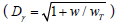versus the normal range of loop sizes of our fermions (10-20~10-23 planck mass) that makes it appear that gravity is weaker than charge. The use of G has hidden the underlying symmetry of the strength of the actions of mass and charge in terms of fractional Planck values, as explained in the foundation paper .

The above interpretation implies that curved motion does not result in acceleration - centrifugal forces are the real ones - and that energy is just as much a vector in action as force, it just requires a different interpretation of ‘energy’, where transformation into a framework of ‘outwards and inwards relative to a point’ eventually leads to greater clarity, although remembering that total energy is always zero for both charge and mass.

Two cases are worth explaining here. The first case is Newton’s bucket which has the liquid contents of a vertically rotated bucket staying inside the container. This is because the force on the liquid is outwards, away from the centre of rotation, and has nothing to do with the mass of the rest of the universe acting on the liquid. When the rotational rate is too low, the liquid will escape because the upward vertical centrifugal force is no longer enough to overcome the downward gravitational force.

The second case is the ‘remarkable’ stability of a bicycle in motion. It is not remarkable once it is understood that the rotation of the wheels causes an outward force, in the plane of the upright wheel, to act on the wheel tending to keep it upright. When the speed of the bicycle, or the rotational frequency of the wheel touching the ground, is large enough in relation to the bicycle mass, there will be a net outward and upward force tending to return the wheel vertical when tilted against the gravitational force trying to topple the bike.

In the case of a particle moving in an inertial reference frame, it is the case that it moves because it already has energy relative to that frame and that energy is another way of describing the force acting within the particle along its direction of travel. The force required to stop the particle is what we describe as equal to the particle’s inertia. It is that if the body already moves, it is because it is acted on by an internal force and has an internal energy, relative to the frame of reference, acting along its direction of travel.

#### Beyond Single Loops

Having looked earlier at the single loops that are the fermions, it is necessary to look at combinations of loops, which have been termed ‘stacks’. The following analysis looks at rotating and balancing symmetry within loops, meaning just where the charges sit within a consistent framework defined by the identity of a similar starting point for every loop. It is not concerned with the magnetic moments of the loops, other than in terms of the spin ±½ of the loop (magnetic moment up or down), or the radii of rotation of the individual meons since these are secondary to the effect of the charges of the meons. As shown later all meons with H mass factors in loops in a stack where all loops are the same size (mass or radius) will rotate at the same inner radius r (1+g/r) and all S mass factors at r(1+k/r) so the larger total charges will have the greatest effects. But it is clear that it is all the mass and charge types that affect the interactions between loops in a stack – the loop deflections (masses), spin energies, s/6 energies, q/6 charges, MM and QQ meon/ meon or meon/anti-meon interactions across loops. The strong force is a mix of these different variants of charge and mass actions along with the loop structure.

Stacks and symmetry – where ‘chemistry’ appears

How do we get ‘chemistry’ is the question. ‘Chemistry’ means the formation of nucleons and atoms and their interactions. With 3-loops, a stack of three loops is required for overall balance for three different 3-fold asymmetric loops. Further consideration shows that 2-loops have 2-fold symmetry. 4-loops have 2- and 4-fold symmetry. 5- loops have 2- and 5-fold symmetry. This ignores the non-symmetric loops, which cannot be balanced by any loop except their own anti-loop.

In order to produce overall symmetric stacks requires that the internal symmetries be balanced. Ignoring 2-stacks for the moment, for 4-loops the only stacks that can be made symmetric contain an even number of loops. The same is true for all even-loops, where there is an even number of pairs in the loops.

What we require for chemistry, at the most basic level in forming atoms, is that the largest-charge symmetric loop can be balanced in orbit around an opposite charge central stack so that the total overall charge is zero. In each loop framework the largest charge-loop is always symmetric, as it must be. Thus it can exist on its own, in a stack of one. But in order to be in orbit, it cannot be only the stack charge that must balance with the orbiting loop, but also the total stack spin must balance with the orbiting loop spin. This is evident from the previous formulae where the spin KE is as important as the mass KE.

Each loop has spin ±½ in the current terminology. In a stack, in order to avoid the possibility of adjacent loops merging, they must stack with alternating spin orientations. Where 2-loops, or other even pair number loops, have the same spin orientation they will form bosons.

To maintain balancing symmetries all asymmetric loops in a stack must rotate at the same frequency – the same size, which should mean same mass except for the fractional effect of fractional charges in the asymmetric loops which changes the observable loop frequencies and the loop apparent masses. So a stack of two will have zero total spin. So will all stacks, where nucleons are concerned, which have even numbers of loops.

What this means is that no even-loop charged stacks can have orbiting maximum-charge loops in stable orbit because the even-loop stacks always have zero spin whilst the maximum-charge loops always have simple spin ±1/2. Only odd-loop charged stacks will have spin ±½ in total and so will balance the spin ±1/2 of the orbiting maximumcharge loop.

The smallest odd-loop number for which spin 1/2 stacks can have orbiting spin 1/2 loops is the 3-loops, with 3-fold symmetry, and these are atoms. This is our matter.

Other odd-loops which can form nucleons with orbital systems are 5- loops, 7-loops etc. But these contain more pairs than the 3-loops and so are less likely to be formed in the first place. The loops that do not form chemistry have no atoms and can have no emission spectra, so are versions of dark matter.

Also likely to be dark matter are the completely non-symmetric 3-loops, which can only balance with their own partner anti-loops, so reducing their likelihood of producing any 3-stacks.

Overall then, chemistry will be most likely with 3-loop systems and the bulk of other systems will not form nucleons or atoms. The larger number of 2-loops formed in preference to our 3-loops will mean that our 3-loop matter will be a smaller fraction of the universe than the sum of the even-loop and other odd-loop systems.

This framework gives rise to multiple loops and composites that react weakly with our 3-loops and their composites. The greatest differentiator will be the charges of many symmetric charged dark matter loops, which will be different to our 3/3q electron charge loops and the magnetic moments of these non-3-loops which appear, from their masses, to be normal matter.

Differentiation between loops with different symmetries will be possible because, as shown later, although the meons will rotate at the same H and S radii, and will have similar radial electric field sizes, their circumferential fields will be different in both size and angle to the radial perpendicular. The meons will be increasingly closer together circumferentially with greater symmetry number and there will be more and stronger inter-meon electric fields.

Stack identities

There are two forms of 2-stack identities, those where each loop rotates in the opposite sense to the other and those where they rotate in the same sense.

Opposite-rotating stacks: The opposite rotating stacks can be formed of any loop pair number provided only that the pair number is the same and they can balance. So a 3-loop can stack with any other 3-loop provided the total stack is symmetric and loops have the same frequency w. This usually means loop and anti-loop, but could be up quark 3-loop with down quark 3-loop, for example. Also possible is 4-loop with anti 4-loop, etc.

One form of opposite rotating stack that is probably present everywhere is the zero spin, zero charge stack made of a 3-loop electron and 3-loop positron. This ‘zeron’ is probably what underlies the quantum mechanical effect of ‘pair creation’. The stack being hit by a particle with sufficient energy can be separated briefly into the two loops – a pair of opposite charge, opposite spin loops seems to appear from nowhere, but were actually always there.

If space is filled with zerons, alongside the existing underlying ZMBHs and acting as part of the viscosity drag in volumes from which they are not excluded (the background), then at every frequency w, centred on every point, there should exist a zeron of apparent energy hw as part of a concentric shell of zerons. Each of the loops will have apparent energy hw/2, and this may represent the zero point energy of that point – although, as mentioned earlier, the total energy of all loops is always zero. This concentric zeron shell framework may also explain physically the Casimir effect, in that the exclusion of shells beyond two parallel plates will result in excess pressure from the surrounding unaffected concentric shells.

Additionally, the denser gravitational clumping effect of 3-loop chemical composite nuclei may result in the preferential location of 3-loop zerons within the same symmetry volumes. This could effectively squeeze the more abundant 2-loop zerons away from the 3-loop gravitational clumps. However, the same zero point energy would still exist across all zerons.

Because of the extra loop identities in loop systems above 3-loops, for simplicity only the 4-loop systems are considered here to represent these as examples of dark matter composites. The total charge sizes for the possible 4-loops are 0q, 1/3q, 2/3q, 3/3q, 4/3q electron charge, positive or negative. The symmetric 4-loops have zero and 4/3q charge. The asymmetric loops are the equivalent of quarks. To form a stable 4-loop 2-stack requires 2-fold or 4-fold symmetry and total charge equal to 4/3q electron charge. This is possible with a 1/3q 4-loop and a 3/3q 4-loop of the same charge for 2-fold symmetry, so this is one form of zero spin 2-stack 4-loop particle that should exist. For 4-stacks, one possible stack combination could be ‘quarks’ of charge 1/3q, -2/3q, 3/3q and 2/3q to give 4/3q overall.

For 2-loop stacks, there is only 2-fold symmetry and two loops are required in a stack. The 2-loop system consists of 0q, 1/3q and 2/3q electron charges. The only way to achieve a stable stack using asymmetric loops is with 2-loop ‘quarks’ of charge 1/3q and 1/3q with the same charge sign, which stack then totals the 2/3q charge of the symmetric maximum-charge 2-loop. These zero spin stacks should also exist, although in a 3-loop environment where q=3/3q electron charge, such a stable 2/3q charged stack will not stably attach to any 3-loop or 3-loop stack to be observed, although it will be deflected appropriately in charge or magnetic fields.

Any length of stack is possible if the constituent loops sum to whole number or zero charges and their overall symmetry is maintained. So considering a proton core of three 3-loop quarks, possible stack additions could be neutrino end caps, quark and anti-quark, electron and neutrino – each of 3-loop and total added spin of zero. The identities of these would be, in order, proton, penta-quark-proton and neutron.

For other sized loops with different symmetries, the same stack lengthening will occur provided the additional loops have the same symmetries.

An extreme example might be the stacking of electrons to form what would be observed to be a heavy electron, with spin 1/2h and integer charge greater than one. This would require extreme pressure to overcome the inter-electron charges. More likely would be the stacking of a single electron with a zeron to look like a heavy electron with total spin 1/2h and a large mass.

Same-rotating stacks: Considering the stacks where the loops rotate in the same sense, there are also two forms here. One is where the loops are a loop and its anti-loop. The other where the loops are different – including different symmetry isomers of anti-loops.

Where the loops are loop and anti-loop rotating in the same sense, the result is a photon with spin 1. Because the frequency of any loop is always measured to be positive, which is the base for all loops having positive observable mass and (even though its energy is zero overall, we measure the split balancing part only) the photon will always be measured to have spin +1.

In the photon, the same forces that drive the chasing of a meon pair round inside a loop also drive the chasing of one meon in the loop towards an anti-meon in the anti-loop, or vice versa.

Figure 2 shows the forces driving meons around a loop and Figure 3 shows the same force action between opposite meon types in a partially merged loop and anti-loop that form a photon.

Figure 3: Photon as partially merged electron and positron loop showing chase forces around the loops and between meons in each loop (loop rotational axia in plane of paper). Each new pair is like an original ZMBH but in motion with in the loops.

The result is acceleration of the loops along their mutual axis of rotation up to the velocity at which the chasing force is equal to the effect of the viscosity of the background field of ZBMHs and zerons.

This maximum speed is what we call the local speed of light, c. This effect can be seen in an experiment  where the acceleration of photons is interpreted as being due to them having positive and negative effective masses within crystals. Actually it is a very clever way of stacking photons so that the chasing from meon to anti-meon internally also occurs externally – as if the photons have become six meon/anti-meons short chains rotating about their axis of motion. This is because a photon contains six of the shortest possible chains – the two loops’ original meons in one loop and the anti-meons in the other loop, and vice versa, acting as new pairs chasing each other –and adding more pairs to the chain can be done by stacking other photons in front or behind the first photon.

Where the viscosity is great, when the ZMBH and zeron numbers are denser near other loops or large masses (planets, stars) then the actual speed of light will be lower than when the ZMBH and zeron field is less dense. But in each location the velocity is the fastest possible and defined as c. That photons travel at a terminal velocity defined as the local speed of light shows that there is internal chasing between meon and anti-meon from loop to loop. This can only be explained if there are negative and positive fundamental masses which chase.

The energy used in matching the background viscosity in order to travel at c reduces the rotation rate of the meons in the two loops that comprise the photon. This is tired light and, apart from at very high rotational frequencies, is proportional directly to the distance travelled by the meons, and thus by the photon, almost regardless of photon frequency.

The spiral distance Dγ travelled over time t by each meon in a photon with frequency w is given by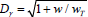where wT is the TAPU Planck frequency. Where w?wT then the meons in different energy photons will each have experienced virtually the same viscosity red shift. The path difference travelled by each meon, comparing a gamma ray at around 1024 Hz and visible light around 1014 Hz, is the difference between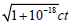and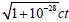respectively – not significant given the uncertainty in the emission point of each.

So the redshift observed in any photon has to take account of this extra viscosity redshift factor. This implies that the size of dark energy may need to be radically reassessed, to the extent that possibly the rate of expansion of the universe is not accelerating at all, if all the excess redshift were due to tired light. Alternatively, the universe, or our successful big bang event, may actually be failing and we are contracting - if the viscosity red shift is larger than the contraction blue shift, or the expansion could be zero and the main red shift is caused by tired light. Overall, it is likely that the observed redshift is a mix of factors including the tired light effect.

This explanation of photons losing frequency to the background has been simplified, as will be explained later, because it is the emission shell (a volume of no-background) that loses the energy and the photon provides it as it skips around the shell.

Using multiple bootstrapping methodologies to measure distances to light emitting objects and comparing these results should allow the existence of the tired light effect to be confirmed and its size calculated within the overall redshifts observed.

The viscosity effect is rather like a very diffuse aether, whose red shift effects can be easily observed only over light years, except that the direct effects are not length contraction in a specific direction, just redshift in all directions. Regardless of the expansional motion of our successful inflation event against the background ZMBHs, because of the velocity of all photons at maximum local speed c the redshift factor (frequency loss) at any point will be the same in all directions.

Photon loops, when measured from the point of emission, can expand and contract in radius due to external frequency transfer, physical interaction or internal frequency balancing between component electron and positron loops, and are stable at any radius. However the fermions, having maximum radii locked in during inflation are only stable at those radii, but can decrease radii, increasing energy, when external motion adds energy to the loops, in a stationary frame of reference. They can also increase in radii as they lose energy due to the background viscosity, explained below.

Where the two loops rotating in the same sense are not loop and anti-loop, the result is a spin 1 boson. These are not force carriers, but are composites likely with high velocities. These also have to obey the total symmetry rules and can be composed of any loop pair number, provided both are the same, so that the total symmetry is stable.

For non-photon loops, the rotation of the loops will be opposed by the viscosity of the background ZMBHs. This should slow the rotation of the loops – effectively reduce their frequencies and what are observed to be their masses. However, it is likely that the continual stacking and unstacking of photon rings provides the extra frequency boost to return a ‘weak’ loop to its preferred size (frequency or mass). In this way, photons and loops lose energy to the background ZMBH and zerons by spinning, moving or otherwise providing them with energy equal to that lost by the action of the ZMBH and zeron background viscosity. The rotational rates which we describe as energy are being gradually returned to the background ZMBHs from where they originated in a big bang event. The preferred sizes for the nonphoton loops are those locked in during inflation. It may be possible to observe the mass loss in non-photon rings by isolating a specific highly accurately measured mass from any light sources and leaving it for long enough – then measure it in the absence of light sources. The rate of loss should be the same as that experienced in the tired light effect for photons, although reversion to normal size when exposed to photons of suitable frequency will be very quick.

Whilst a photon is more likely to be a single electron and positron ring rotating together, it is possible that neutrino and anti-neutrino, or quark and anti-quark could also form a photon double loop. And it is even more likely that longer stacks of photons could form because each pair partially merged across two loops could be similarly merged with another photon of the same size. The actual length of a stack of photons may be the wrong way to describe such a photon. A better description may just be the number of double loops presents.

It is even possible that a stack of separated photons, each chasing the one ahead as if it were a chain, could catch onto its own tail. Then the ‘mass’ of that super-loop would depend on the rotational frequency of the photons as they travel around the super-loop. Given that the photons would be travelling at local c, the ‘mass’ of the super-loop would be the adjusted-Planck mass regardless of how many photons formed that super-loop.

#### Strong and Weak Forces

So far the description of stacks has not directly mentioned strong forces, only forces or energies due to charge and mass. That is because there is no different strong force. What looks like a strong force is only the actions of charge and mass, both fundamental and normal, by loop systems when close together. At the intra-stack distances, the actions are largely from meons in one loop to meons/anti-meons in the adjacent loop in the stack. At inter-stack distances, where two stacks are similarly aligned adjacent, the same is likely also the case. It is the loop nature of the composites that introduces preferential separations. At stable intra-loop and inter-loop distances the forces of attraction and repulsion due to charge and gravity (of equal strength for equal adjusted Planck fractional values, and via their various types) are balanced.

The weak force is not mentioned because it is the effect of the physical replacement of one loop in a stack by another. For example, a neutron stack (5 or 7 3-loops with a core of three quarks and caps of neutrinos and an electron) can have its electron loop knocked out of the stack by the appropriate size impact of an incident neutrino, resulting in a proton. The effect is random and depends on the density and energy of incident neutrinos.

The reason why the electron and neutrino can exist within a neutron stack is because they are symmetric already, and have hidden 3-fold asymmetry, and because their components, the meons and antimeons, are large. The actual physical size of the loop, which we call its mass, is not of consequence as to whether it can exist inside a nucleus or not.

However, there is a preferred size of symmetric loop to enable better stacking, so the electron swaps frequency for magnetic moment. As shown by the symmetric charged members of loop families, the electron family can change physical size/mass in exchange for magnetic moment between preferred family sizes. Magnetic moment is just another way of describing the mass or charge energy of the loops.

Interaction between separated loops is complex. For every loop there is an extra chasing force towards or away from, another loop due to mass-chasing, which would not be expected. The effect may be masked by charge interaction, or be zero if the loops are at unchanging separation, as in a stable orbit. The effect is dependent on the difference between the two loop frequencies, the initial direction of travel of the two and the mutual relative orientation of their planes of rotation. Mostly the effect is to introduce an effective vibration of the loops as the chase direction between meon/anti-meon changes. But there may be an overall net chasing driving the meon/anti-meon, and their respective loops, along the lines between them. The effect is most efficient and obvious in the case of neutrino/anti-neutrino or electron/positron comprising a photon.

#### Where the Electron Got Its Size from – Inflation

We probably owe our existence to the size of the electron. Had our original successful big bang been less inflative, the electron would have been physically smaller, so of larger mass, and our inflation event could have failed.

In the great unmerging event that preceded our big bang, where chains formed, broke and eventually formed loops, at some point those loops interacted physically to hit each other. The result was a sudden drop in speed of some meons with a resulting huge increase the physical size of the loops, of which they were components, to conserve angular momentum h for the meons in the loops (when considered in DAPU units and ignoring h± , as explained later).

In a three dimensional universe these ‘inflated’ loops would have been quickly aligned along three axes to provide three different rates of inflation, a different one along each axis. Even a loop being off axis would become on-axis with sufficient inflation, producing flatness and standardisation of fermion loop sizes with preference for remaining within the three family sizes. Three dimensions are needed as a minimum, because it is not possible to overlap or merge objects of only two dimensions, and more than three are not necessary.

Given a suitable mix of the amount of inflation along the three axes, it is possible to produce eleven fermion masses in the right range out of the twelve we observe. So four fermions and three dimensions of inflation probably represent all that is needed to produce the three fermion families with their physical sizes/masses.

It is possible to assume that inflation only affects the loops where meon and anti-meon are not merged – single fermion loops and not photons where each meon/anti-meon pair are effectively a reformed ZMBH. This means that the single fermion loops would inflate, but not the photons.

The effect would be that the single fermion loops would inflate so that their centres were further away from the origin of the initial unmerging event than the chains which formed photons at near Planck energy. This would allow the fermion single loops to be ahead of the photons in expansion until the photons caught up. At that point, the energy (frequency) of the photons interacting with the fermions would result in the formation of fermion then nucleon stacks and the subsequent reemission of the photons to continue their outward expansion, some now ahead in the expansion of the fermion/nucleon mix.

Expansion is used here to mean simply the outward motion of the components of the initial inflation across the background of generally stationary ZMBHs. The vector nature of energy, as represented by half the zero balance, means that a symmetric expansion also has zero energy of outward motion in total.

#### Failed Inflation Events and Chain Stars

The paper sets out in part to provide a logical framework for using the simplest possible assumptions to build a universe with the laws, properties and symmetries which we observe. These assumptions do not lead to anything which can exist outside our universe, since everything that emerges is made of components from within our universe.

This does not preclude different inflation events. However, these will be within our universe. So if there is only one size of building block, no expansion of space can be taking place, part of what we see as expansion is red-shift in photons.

Where these events failed, they may be observable and where successful, they also may be observable, but not yet recognised, or beyond our observable horizon but still part of our universe, not any form of external multiverse.

Where multiverse and black hole event horizon models are some of the ‘most speculative’  physical theories, what is proposed here is considerably less speculative, but is still speculative from a completely different viewpoint to those normally used in multiverse or black hole speculations.

In this section the normal understanding of ‘energy’ will be used in the explanation, although, as previously explained, it should be understood that for every rotational or motional mass energy considered, there is an opposite balancing charge energy. The reason for any change is the different way in which charge and mass energies interact with themselves, rather than each other, from loop to loop or meon to meon in different loops. So the total energy released in unmerging, inflation and expansion is zero. What is retained is the angular momentum of each meon and anti-meon which, in total across all meons and anti-meons, will also total zero.

The inflation of the loops, in the meon speed drop, releases huge amounts of energy (frequency) from the loops which then drive expansion away from the source of the initial collisions by speeding the resultant larger radius (smaller mass) loops outwards. The question is how much is released. If the loops that emerge from inflation are large enough in physical size (small enough in mass/energy) then the expansion will more easily drive the loops outward and their mutual gravitation will be too low to oppose that expansion.

The release of energy is the difference between the small size, approximately the Planck-energy, at which loops formed from the initial chains, and the eventual post-inflation large sizes (small energies) of those loops – the masses of the fermions. This factor for us is a maximum around the inverse of the mass of the electron in adjusted Planck units, or of the order 1023. The time taken for the meons in an electron loop to adjust loop size from around Planck energy to the electron observed size at velocity c will be of the order 10-29s, the path being across the diameter of the new larger radius electron loop at velocity c.

If the speed drop is not large enough in the physical interactions between loops, so that the resultant inflation does not release enough energy for expansion, the loop sizes will be small and their masses large. Here the energy for expansion may be insufficient eventually to overcome the gravitation of the resultant larger mass loops. This is a ‘failed inflation’ event, where the balance between inflation and expansion was wrong, and the loops will collapse back towards their starting point under the effect of their large mutual gravitation.

A failed inflation event does not lead to a big crunch though, because the initial unmerging of ZMBHs to form loops has changed the environment. When the loops fall back in to the centre of their expansion, the result is likely to be a ‘chain star’ where the loops eventually become broken by the strength of gravity into pairs again. Within the chain star are the same processes that occurred after the big bang unmerging event, but without the formation of the first loops at the smallest possible size (greatest energy).

The loop size (mass) and spin energy are both reduced to zero as the loop is stretched, by differential strength of action of gravity across the loop, and its frequency reduces as it descends into the chain star and is finally broken. The charge stays with the pairs in the chain. Inside the chain star, the entering chain will get broken, mixed, form, reform continuously until a photon double loop of sufficiently large energy (smallest size) escapes. As it does so, it will lose a lot of its energy. This is how a chain star evaporates and where it gets its temperature from and how the previously entering loops’ information escapes in a different form.

A chain star chaotically makes and breaks the loops that fall into it. It emits only symmetric loops in the form of high energy photons that have enough energy to escape the gravity of the chain star as low energy photons. Chain stars are what we describe as black holes. The chain stars reduce in physical size as they successfully create high energy loop/anti-loop pairs that are emitted as photons . The photons do not need to be 3-pair size and will be any number of pairs, although 2-pairs will dominate as dark matter photons.

Failed inflation events themselves will have gradations of end results. For the ‘heaviest’ loops formed, it will be difficult for the loops to escape mutual gravitational collapse and a chain star will be the result. But for less heavy loops, maybe near in size but not as light as our fermions, the result could look like a galaxy with the results of expansion almost balanced by the effects of mutual gravitation. So a scale of failed inflation events could exist, dependent also on whether the initial inflation event contained any rotational effect. This latter effect may make a galaxy appear to have stars with greater velocities than normal mechanics would expect and might be viewed as needing surrounding dark matter to exist.

Additionally, it is not clear that a failed inflation event with a slightly larger ‘electron’ mass would allow its larger scale atomic emission spectra to be differentiated from either a faster moving galactic source or one at smaller distance, given the accuracy of spectral observation. As the fermion mass sizes increase with greater failure effect, at some point the difference should become observable.

The photon emission spectra from such a 3-fold symmetric system with greater ‘electron’ mass would be proportional to the ‘electron’ mass, it would appear to be more energetic and so blue shifted when viewed in isolation to the other redshift components.

Assuming that unmerging events happen randomly in the background ZMBH space, then many black holes or galaxies will have been formed before our successful inflation, and also since then. They are embedded in the background which is the foundation of our universe. What we see as an excess of early black holes, or the early formation of stars or galaxies because of those black holes , may be just a reflection of the rate of failed inflations. If we could differentiate between failed inflation black holes or galaxies and subsequent black holes and galaxies formed from excess density gas clouds, we could make an estimate of the rate of unmerging events.

Another aspect that may allow the accuracy of this framework of black holes as failed big bangs would be the discovery of unarguably conjoined galaxies or black holes , where the redshift of each is markedly different without any alternative explanation. One redshift would represent the relatively stationary background mixed with tired light from it, whilst the other has a different redshift representing its local rate of expansion mixed with the same tired light factor as the first. Finding a number of these would allow the tired light factor to be separated from the overall redshifts. Effectively the lower redshift represents the partly stationary background tired light factor and the difference will be mostly the motional expansion redshift. The number of such real conjoined systems within a given volume would provide an estimate of the rate of failed big bangs.

The universe cannot be expanding due to inflating space between galaxies because that would imply that the base unit of space was expanding. And that cannot be the case since all ZMBHs are the same adjusted TAPU Planck size, so expansion is just motion versus the background of ZMBHs.

This represents a mixture of both the big bang and steady state theories of the universe. The steady state here is confined to random big bang events that either fail or succeed, instead of the continual creation of matter between expanding space. The result is that the universe could be far older than our inflation event suggests and could be much smaller. Furthermore, earlier failed inflation events could have formed seeds for later gravitational accumulation during our own big bang, providing a quicker route to star and galaxy formation.

Black holes also have another two roles in the universe. One is that they convert asymmetric loops into symmetric ones, and in the process reduce the amount of loops showing mass within the universe, so reducing the gravitational effect of all loops with observable mass in the universe. This has two effects. One is that the expansion of the universe may accelerate as the overall gravitational effect reduces, which might look like the acceleration of expansion or dark energy . The other is that there will appear to be more photons than would otherwise be expected with increasing discrepancy over time . This is because more bodies, symmetric and asymmetric loops will have been swallowed by the black holes and turned into exiting photons. The symmetry generating effect arises as matter, anti-matter and dark matter loops are broken when they enter the black holes and they can only re-emerge as symmetric photons, having sufficient energy to escape, as mentioned above.

The current quantum pair creation explanation for emissions from, and evaporation of, black holes is not possible in this framework. Zerons, which underlie pair creation, already exist and become observable given sufficient impact. If zerons were close to a black hole, as if acting as an event horizon, they would be stretched and swallowed by the hole. So pair creation as the primary source of black hole emissions is not likely.

The second other role of black holes is in dark matter transformation. The breaking and reforming of loops shifts the ratio of dark matter to normal matter because the same probabilities of reforming chains of different pair number will make many more 2-pair photons than 3-pairs. Dark matter photons will be preferentially formed and emitted, although they will not have any effect on the charged lepton’s g-2 value since they have the wrong symmetry .

So the current ratio of dark matter to normal may have been significantly changed by black holes over time, especially if the galaxy under observation is a failed inflation event from before our own big bang. The dark photons may either not be observable to our 3-pair detectors or may have been broken into loop and anti-loop amongst other dark matter loops. With enough black holes and galaxies emitting dark photons, there will seem to be no reason for the extra ionisation of gas clouds .

The consequent effect of dark matter transformation would be to reduce the amount of normal matter surrounding the black hole and leave the galaxy looking as if it does not have enough matter to retain its matter in orbit around itself.

So it may be that the amount of inflation sufficient to produce a small enough electron, and our other fermions, is what separated our successful inflation from a failed inflation. It is also possible that there is a range of possible small loop sizes that could be successful in overcoming gravitational collapse, but outside the parameters necessary for successful chemistry. Maybe our electron size is the lowest limit for both successful inflation and successful chemistry.

It is possible that the symmetry of a failed inflation event affects the final outcome. With perfect symmetry there would be no rotation of the expansion away from the event and the resulting deflation would be rotationless. The result could be a simple black hole. However, an asymmetric expansion could involve the rotation of the whole number of loops formed, which would delay the deflation and possibly enable the formation of a rotating galaxy.

For loops entering a black hole, the breaking of the loop is where time stops for that loop. Only loops have our time and our time only formed when loops did. For different big bangs, successful or unsuccessful, normal or dark matter, the only time the loops know is their own rotational rate. In such a system, without overall rotation of the universe, all centres of rotation are equal and there is no preferred central frame of reference.

#### Laws of Physics are Identical – Always and Everywhere

The loop sizes are the only variables in the chemistry of the universe, because the one-sixth charge will always be the same since it represents the energy required to unmerge all foundation ZMBHs into meon and anti-meon pairs.

These failed inflations are within our universe. Using the ZMBHs as the only source of building blocks for composite loops, it is not possible to have any universes ‘outside’ ours. There may be other unmerging events, inflations and big bangs, but they are using our foundations, within our three dimensions. Other successful inflation events may be so rare that there have been none, could have occurred beyond our visible horizon or not have been recognised as such yet.

As shown above, any inflation events, failed or otherwise, will produce 3-loop systems with potential for chemistry and dark matter with the same approximate relative starting ratio of matter to dark matter as ours. What will differ is exactly what size the loops end up as. This will affect the sizes of atoms, photon emission lines etc but not how gravity and charge work, the size of one-sixth charges and three dimensions. All underlying physical laws will be the same in all ‘universes’, if that is what inflation events are called, whilst they evolve to be either failed or successful.

The idea that physics breaks down within black holes, forming singularities, is wrong and is based on the idea that energy/matter is packets of amorphous waves or particles.

If the foundation of loops is TAPU Planck size meon and antimeon, then they are the densest particles possible in the universe. There is no possible assembly of particles which could break meons apart. So a black hole, or chain star in the preferred description, is far less dense than any meon. And if the universe is populated by and constructed from unbreakable meons, then physics does not break down anywhere. And that is why the foundations of the universe, as merged volumes of meon and anti-meon, are called ZMBHs – zero mass black holes. Everything we are made of and can observe is composed of the densest micro black holes, and massive black holes do not contain singularities.

The question of whether there is an event horizon around a black hole is the wrong question. The loops are stretched until they break back into chains as they approach the massive black hole. However, different loops break at different distances from the black hole, so the break point is smeared, without a specific horizon and depends largely on the strength gradient of the gravitational field – a factor dependent on the size of the black hole. The larger the size, the lower the gradient and the more smeared the horizon.

And the question of how time is affected as the hole is approached can be understood – as the loops elongate on their way towards the black hole, due to the differential gravitational field on each meon in the loop, they slow in frequency and their own time slows until it ceases to exist when the loops break. For both the loops and any observer of those loops, the time/energy of those loops is no longer observable.

When the loops break, the identity of the loops is lost and the angular momentum of the meons in the loops has been transferred to the hole. That internal momentum was what is described as the mass and spin of the loop, so the mass and spin are transferred, as is the overall loop charge.

What happens inside the hole is that the pairs in the chain, that was a loop, retain their twists and if they later exit the hole as part of a photon, they will show the same fractional electron charge that they have always had. So although the loop identity disappears, the later emergence of any pairs from that broken loop will see some of that identity reappear, although within a different loop. So information is conserved, but at a lower level than the loops.

Also worth mentioning is the lack of need for any Higgs mechanism. Mass is the size of the loops and is a measure of their frequency of rotation. The Higgs is just a boson composed of an even number of loops. The loop framework does not require anything further, although when producing a framework for the successful modelling of the magnetic moments of the loops and nucleons there is a further relationship between the size of magnetic moment of loops and their masses and charges, as described later. There may be many more matter bosons yet to be discovered at high energies, but they are simply longer stacks.

#### Hierarchy of Zeroes of Total Energy

What drives the states of matter that we observe? It is a preference for having the least energy possible in the simplest state, using ‘energy’ here in its accepted meaning.

The state of the stationary ZMBHs could be said to be a quadruple of zeroes of total energy (‘ZOTEs’). Not only do they have no motion if considered in their own frame of reference, but for fundamental energies, each charge energy is balanced by an opposite charge energy, each mass energy is balanced by an opposite mass energy, and each charge energy is balanced versus a mass energy. Even if they rotate, spin or vibrate, these motions can be reference framed away. This is the preferred state for two merged meons. Once unmerged, they would prefer to get back to that ‘perfect’ state.

When unmerging a ZMBH, two ZOTEs are lost and each meon or anti-meon is left with only a balance of charge versus mass energies, although it has added a ZOTE for one-sixth electron charge energy versus mass twist energy. The pair is driven to regain its lost ZOTEs, due to charge balancing charge and mass balancing mass. So they chase each other to try to remerge.

The formation of a chain does not change the situation for the pair, but the chain latching onto its own tail produces another ZOTE. This is the balancing of the motional energy of the pairs with their spin energy, their mass and spin, from the point of view of the loop combination. This could be reference framed away by considering the loop to be stationary and then the meons would be missing their drive to remerge. So the loop framework conserves that remerging drive and adds the mass versus spin ZOTE.

The next level is the stacking of asymmetric loops, which is a form of ZOTE in that the result is stable, so the imbalanced energies are hidden. However, the stack will have an odd number of loops in our threefold symmetric matter and so the next ZOTE will be to balance out the spin energy of the stack by forming a stable composite with a suitable symmetric loop in orbit. Additional charged nucleon stacks in a core will require additional loops in orbit and any lack of the latter will drive the formation of compounds with other atoms which have an excess or deficit of these loops in orbit, as another ZOTE is formed.

Even at gravitationally dominated levels, the orbiting of two bodies will prefer the formation of a ZOTE – it is just that so far the spin KE of the loops in the orbiting bodies has been not been considered correctly in the energy equations. This means that the zero total energy involved in being in a gravitationally stable orbit has not been understood.

Whilst photons are considered as having energy, this composite is again simply another form of ZOTE. For an electron and positron, the mutual formation of a photon provides almost the original quadruple of being in a nearly reformed ZMBH. Each meon and anti-meon, merged across both loops, has the quadruple ZOTE, but additionally there are the twist balance and the mass versus spin ZOTEs present. For the loop overall, its motion produces another ZOTE because all the motional energies balance, but this could be reference framed away in any case.

It can be said generally that all interactions are preferentially driven towards ZOTE states in some form. Where particles or systems are not in ZOTE states, they will not be stable until they reach such states, whether by motional, potential or other energy gain or loss.

It is not clear how pairs could eventually remerge into the original pure quadruple ZOTE state because they have the additional twist ZOTE, having gained it on unmerging. However, this does suggest that the hierarchy of ZOTEs may partially underlie the second law of thermodynamics. The direction of progress from ZMBH to gravitational system may be from low number of ZOTEs to higher number, perhaps with increasing entropy in each ZOTE number that the particle or system has, with the reverse direction requiring an energy input.

Interaction between loops close enough together is by physical interaction or by the loops’ mass and charge fields that transfer loop frequency from one loop to another. The total frequency of the loops involved, adjusted for potential, magnetic or other positional energy effects, will remain the same. This is the basis of conservation of energy.

Another method of conservation of energy is the change in loop size/frequency of electron into muon, for example, balanced by the change in magnetic moment, gravitational, kinetic or other energies.

Because loop frequency is also a measure of the effective temperature of a loop, the inability of a lower frequency loop to speed up a higher frequency loop provides another physical the basis for the second law of thermodynamics.

And underlying all motion is the loss of frequency by all loops when they move across the background viscosity.

#### How to Uncover the Dark Matter Framework

Amongst the dark matter particles should be opposite sense rotating variants of symmetric 4- or other even-loop zero spin particles which when separated would have charges of 4/3q electron charge, and the same for spin 1 bosons split apart. The magnetic moments of non- 3-loop particles will be different to 3-loop moments, which will enable their identification.

Another proof is in the photons emitted by black holes, whether from failed inflations or otherwise. The photons emitted must be symmetric, but can be of any loop pair number. But it is only the 3-loop photons that will stack successfully with electrons to boost them between orbitals because they have 3-fold symmetry. So photons that arrive at detectors with suitable energies but which do not boost electrons, or that when separated have symmetric and stable ‘electron’ and ‘positron’ emerge with fractional electron charges, will show that the loop hierarchy is correct.

It is also possible that other successful inflation events have not yet been recognised as such. The failed ones will probably be embedded within other matter systems by now. But there may be examples of large black holes, chain stars, which have no surrounding matter and no other explanation for why they exist alone, but could be observed in the search for MACHO objects .

#### Time and Quantum Mechanics

Quantum mechanics has not been mentioned much so far, but the identification of fermions as loop entities underlies their wave nature. So loops are the basis for all gravitational interactions and are the simplest form of quantum gravity, in that each loop is its own quanta of gravity.

All loops carry their own time with them, as the inverse of their frequency. In a non-rotating, or slowly rotating, universe this means that all loops have frequencies (their masses) which are absolute in the framework of the universe, but which are relative to each other. However, in the framework of the ZMBHs, there is no observable time because the loops, which we use to observe, cannot observe ZMBH rotation or vibration. So there are two different motional types of time in the universe. One which the loops exist within and one for ZMBHs. The latter time may also be as relative between ZMBHs as it is for loops relative to each other. The only thing that can be said is that the viscosity of the background ZMBHs and zerons, where they exist within a volume, effectively transferring energy to them from the loops, provides an unambiguous arrow of time. No event can be reversed because all loop components in the event have lost energy to the background, so the loop has lost frequency.

There is one further level of time, but this is the equivalent of either a stationary ZMBH space where there is no motion, rotation or vibration or the complete absence of background ZMBHs within a volume. This is the complete absence of time – in the latter, no viscosity affects the motion of loops and so there is no velocity limited to c. This state is what seemingly underlies most quantum mechanical effects. The absence of ZMBHs from a volume is effectively outside our relativistic universe, but part of the whole universe.

The emission of a photon should actually be considered as the creation of an expanding spherical shell within which the photon moves outwards from its source. The shell is an absence of ZMBHs within which the photon can move instantaneously and randomly within the shell to any point in the shell, called ‘skipping’. It is the shell that at all points moves against the ZMBH viscosity outside, like the bow wave of a boat leaving a depression in its wake, with the photon as the indicator of the loss of energy against that drag. When the photon is observed, the effect of all the stars and galaxies over which that portion of the shell has moved is reflected in the photon.

The instant before the photon is observed it could have been situated at the farthest point of the shell from the observer and then skipped around the shell to be observed, travelling at above c. If it did not skip into the right place, it would not have been observed. So the path of a photon from emission to an observer’s eye is a completely random walk around the expanding shell with the only fixed points at emission and observation.

For example, whether one or two slits are open before a photon is observed hitting a detector does not matter to the photon until it is actually observed. One gate can be closed after the photon should have passed through, but the result will be what the shell experiences .

The non-local effect provides what is called superposition of particles. Here it is not a shell, but a tunnel without ZMBHs or zerons, through the background ZMBHs and zerons, which links the particles. The particles skip randomly and almost instantaneously between the tunnel extremes because there is no viscosity in the tunnel and the observation of one particle immediately destroys the tunnel and locks the other particle where it was at that instant. The effect appears to be superposition because the particles are exchanging position through the tunnel too frequently to observe. The entanglement of single or multiple loops opens up a ZMBH-free tunnel between the entangled entities and is the basis for non-locality.

Another example is the orbitals of electrons around atoms. Here the shell, within which no background exists, may be in multiple pieces around the atom, but the electron can skip within the shell parts without viscosity and instantaneously from any point to any other point within the shell parts. There is no time spent in motion between the shell parts and the electron can be anywhere in the totality of the shell at any time. It is possible, when considering the same sort of split between expanding orbital shell and loop position for photon travel, that it is the whole orbital that retains the action of the charge within it regardless of the actual instantaneous position of the electron. This could be experimentally investigated for non-spherically symmetric orbitals, although the extremely fast skipping would make separating the two distributions difficult.

It is possible that the charges initially generated by the twisting of the meons against the background would no longer be generated in tunnels where there are no background ZMBHs. This would allow a loop and anti-loop to have no charge effect of each other as they move along the tunnel. Possibly the meon twist energy would be balanced by translational motion along the tunnel until the tunnel is destroyed and the charges re-emerge due to the background flooding in. Or possibly the charge energy may be used instead to keep the tunnel open until the system is disturbed.

Another possibility is that separated parts of a whole orbital are joined by tunnels, although this would mean that the electrons could exist in volumes (the tunnels between parts) which they should not from a probability analysis. This is not the preferred solution, but the alternative is that orbital parts are joined in some higher dimension, which is also not a supported solution.

Another description may be that the superposition of the two loops that comprise a photon create a bond between them that could be seen as a tunnel joining them through the background, keeping the background out of the way, so that they can move along the tunnel, swapping places randomly at above light speed, because there is no background to slow them. The distance we measure between the two loops does not matter because the tunnel effectively means that they are always in contact, because their travel time is almost instantaneous.

#### The Action Modes of ZMBHs

The framework here does not require bosons as force carriers. Instead the background ZMBHs transmit forces in five different modes of action across space. The five ‘space’ actions of the ZMBHs can be split as follows:

A Magnetic Flux Line - The ZMBHs, as a pair, are partially unmerged and spin about their symmetric axis of rotation, without any external motion of the pair. The field between the meons is both electric and gravitational, but since there is no relative motion between the pair, external gravitational chasing does not occur, they are in balance. The sum of the mass-like part is zero, as is the charge-like part, and all that remains is the rotating electric field. This rotating electric field generates a magnetic field along the line of the rotational axis which does not extend far beyond the ZMBH pair. This is one building block of a flux line. A continuous line of these ZMBH pairs will form a complete flux line, started by the magnetic field produced by a loop or any magnet and finishing as a complete circle on the opposite side. The rate of rotation and ZMBH pair separation define the strength of the field at that particular point.

B Electric Field Line – attractive charges - The ZMBH pair is partially unmerged and is not rotating or moving externally. Since it is not rotating and the meons have no relative velocity, there is no magnetic field or any external gravitational chasing. The electric field lines up between two opposite charges, with alternating ZMBH pairs, each separated or partially merged with the next in line, forming an electric field line.

C Electric Field Line – repulsive charges - The ZMBH pair is partially unmerged and is not rotating or moving externally. It does vibrate along the line between charges, centred on the stationary meon with charge opposite to the external charges. The same-charge meon vibrates through the opposite-charge meon and its slight excess energy relative to the opposite-charge meon, due to its relative motion, is enough to maintain the pair in position. It is also possible that the moving meon orbits around the non-moving anti-meon, or vice versa, which would maintain meon to anti-meon separation and avoid external gravitational chasing between them. The vibrational or orbital frequency would depend on the strength of the field at that point and the orientation of rotation would have to alternate along the chain to keep the average total magnetic field zero.

D Gravity – Because the loop has zero energy overall due to mass or spin energies, it is the meons and anti-meons in the loop themselves which interact with the ZMBHs. Since an isolated meon is the densest particle possible, it will represent a maximum action of gravity (or charge). A positive meon in a loop will be moving at the loop rotational velocity and the negative meon in an external ZMBH pair will chase it, with its own partner doing the same to it. This will extend out in an external chain-like structure with alternating meon and anti-meon ZMBH pair, latched onto the meon in the loop. The meon-to-antimeon separation/merger will represent the strength of gravity at that point – a form of deflection acting on any other loop that approaches. An external chain based on a loop anti-meon will have the reverse orientation, although at great enough distances the difference may not be observable. This action is called dragging – where the meons and anti-meons in the loop drag chains of ZMBHs around as the loop rotates. This whirlpool-like effect extends out to an influence distance, beyond which the swirling no longer enables one loop to identify the orientation of another loop, only its frequency of rotation which represents its mass.

E Spin – The ZMBH interaction is identical to that for gravity, except that it is the charge of the loop meons and anti-meons which attracts the ZMBH pair. The size of effect is the same and the chains formed are the same. However, at separations greater than the interaction distance, because the orientation information is no longer available, the interacting loops cannot react to each other’s spin energies. Where the gravitational chains provide a continuous gradient of gravity along the chain towards the loop meons or anti-meons (attraction), the same ZMBH chains due to charge provide no overall charge gradient along a chain. So the interaction of loops due to spin is because physically their whirlpools interact.

The action of two whirlpools with similar rotational orientations is to combine, whereas the action of two oppositely rotating whirlpools is to repel, both when inside the influence distance. The relative angle of the whirlpool planes of rotation will also affect the strength of interaction with a minimum when the planes are at right angles. Although the overall charge along each chain is zero, there will be an alternate electric field extending outward along the chain from each loop meon and anti-meon which will be non-zero and larger closer to the loop meon or anti-meon.

This explains why the spin-spin energy interaction is very small in large bodies with randomly oriented spins, but is large in aligned spin systems. It also shows that the kinetic energy of the spin energy in any motional system needs to be considered in exactly the same way as the mass kinetic energy. And that the size of mass energy and spin energy is the same in any loop.

#### The Electron

The next level up from single fermion loops was considered earlier, as were the wider implications at the largest scale. Now the foundations of the composite nature of fermions and the basic structure of loops can be analysed in more detail.

As shown earlier the two sets of equations governing the motion of meons around a ring can be shown as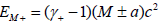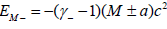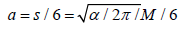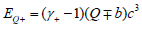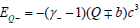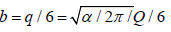We set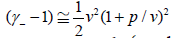at v<c and v(1+p/v)=r(1+g/r) w for the positive meon, with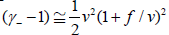at v < c and v(1+f/v)=r(1+k/r)w for the negative meon. Here v and r are the simple values that would be the case if there were only M energies, not the additional a and b mass and charge twist energies and the factor p, k, f and g are small changes in the velocity and radius of rotation respectively of the meons.

Because a meon pair, as considered here, must rotate at the same frequency w, it can be shown that k/r=f/v and g/r=p/v.

So now the four equations become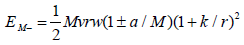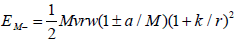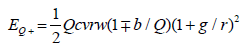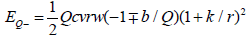Mass and charge currents

To find the part of the magnetic moment due to the motion of the charges around the loop requires the simple formula relating the actual charges and area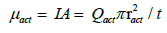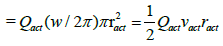Comparing this result with the four formulae shows that the equations for μ are contained within the energy formulae, using the appropriate actual values for each meon.

Considering firstly the symmetric electron. It is composed of three identical pairs of meons. In order to have an overall negative charge of size equal to one electronic charge, it must have all b of negative charge. This means that all a must be positive. We can consider just one pair and multiply the result by three.

The charge current for one pair can be calculated as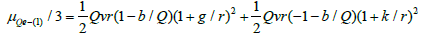This is not the only contribution to the magnetic moment because there are also electric fields, in the frame of reference of the rotating loop, across the loop to the opposite meon and both towards the next in line, and from the following meon. Figure 4 shows the relative positions.

Figure 4: Electron loop with charges _Qa at Ra and + Qb at Rb each from center of rotation O.

Similar to the charge current is the mass current, which for the three electron pairs will each be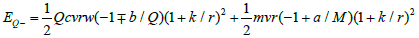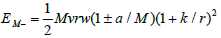This is not to say that there is a magnetic moment due to the meon mass energies in motion, only that all equivalent motions of mass or charge can use the same equations. If there were no a/M energy factor, then k and g would be zero and the simple equation for one meon would be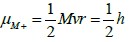Note that the relative radii in the meon equation have swapped relative to the charge equation, as has the sign of a/M.

Because a/M and b/Q are both ratios and of the same size, they can be simplified to |a/M|=|b/Q|=j.

Since the mass angular momenta for each meon in a loop must be the same, this means that the mass current for the loop must be zero, so the mass current equation for three pairs becomes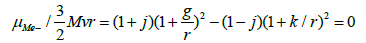The solution for this relates k and g but does not pin down either yet. Replacing (1+j)=H, meaning ‘Huge or larger’ and

(1-j)=S, meaning Smaller, the relationships are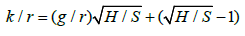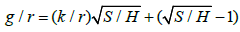The use of H and S is important in the simplification of the possible combinations of different radii of rotation of the meons within loops.

A possible simplifying assumption: Although the value of h± could be different to h, as has been used so far, it is also possible to consider that each meon, even when at (1+g/r)r or (1+k/r)r has in total still only h angular momentum, as it would if there were no a/M energy factor. So individually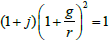and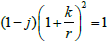would be the case.

The result would be that g must have a negative value, representing a distance inside the ‘no a/M’ radius r and further that the charge current equation for one pair would now become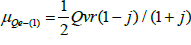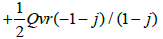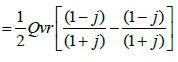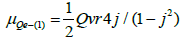And for three pairs gives the pleasing result that the effect of the offset rotational radii is slightly more than double the effective q charge current. Instead of six q/6 charges providing just one q charge current, the offset adds more from the meon fundamental charges, since the effect is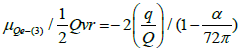This does not eliminate the need to consider other values for h±, and the other effects providing magnetic fields, but is interesting in itself, because it pins the angular momentum of every meon, regardless of rotational orbit, at h. However, the possible alternate values of h± will be considered in the rest of the paper.

If the value of h± were equal to h, it would follow that the same formulae would apply to all charged lepton families for circumferential charge currents, meaning the same adjustment factors k and g relative to the different radii of the electron, muon and tauon loops, although each would be different absolute adjustment distances.

Scale of magnetic moments: The relative scale of this moment needs to be considered because the calculations here are all based on internal loop velocities, radii and charges, whereas the usual meaning of the magnetic moment of the electron relates to the external velocity and radius of an electron orbiting in a magnetic field.

As shown, the charge current for three pairs in the electron can be recast in the form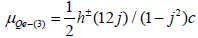where h± means the value of angular momentum appropriate for the radius of rotation of the meon under consideration, which may not be h.

For a ‘standard loop’ where there would be no twist energies present then j=k=g=0 with h±=h. The standardised magnetic moment of any loop would be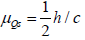and the electron standardised anomalous factor due to charge current would be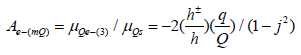To convert this into the orbital framework requires the multiplication of this factor by the ratio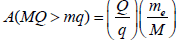So that in terms of an electron orbiting at velocity vorb and radius rorb with mass me, with h=mevorbrorb and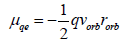Then the value of standardised internal electron charge current as an anomalous magnetic moment in the usual orbiting framework will be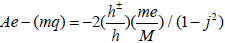This value is too small to affect the 12 decimal places of current accuracy of the electron anomalous magnetic moment. The case for the electric fields is analysed below.

Mixing rotational radii in loops: For each meon pair there are only four different outcomes of H and S for the meon mass M as shown in Table 1, reverting to using a and b to define the different energy components. The first outcome H+ S- is called T1 and has the H+ positive mass meon at radius (r+g), the inner radius of the two. So it has a larger positive mass factor but rotates at a smaller radius. For the charge side, this same meon has the smaller charge also at the smaller distance (r+g). So this pair may have a balance of mass current but they have an excess of negative charge current since the larger charge orbits at the larger radius. So this pair of T1 type represents one-third of the electron loop. By symmetry, the type T2 pair makes up one-third of the positively charged positron.

Type T3 has a mix of charges on each meon of the pair, with both meons showing H sized mass current. These will rotate at the same (r+g) radius when they are mixed in with a loop that requires equal angular momenta, but otherwise if all three pairs are T3 , in a symmetric neutrino, they can take any radius.

Type T4 has a mix of charges on each meon of the pair, with both meons showing S sized mass current. These will rotate at the same (r+k) radius when they are mixed in with a loop that requires equal angular momenta, but otherwise if all three pairs are T4, in a symmetric neutrino, they can take any radius.

It is by mixing types within a loop that the quarks and asymmetric neutrinos can be made. The 20 mixes of types that are necessary to form leptons and quarks are shown in Table 2.

What is apparent is that in every loop, other than the symmetric neutrinos, there are only two radii at which meons rotate. All meons with H as the mass factor will rotate at (r+g) and all meons with S as the mass factor will rotate at (r+k) when (r+k)> (r+g), and it is possible, as shown earlier, that g could have a negative value.

Although this simplifies the possible structure of each loop, it does require each meon and its relationship with all other meons in the loop to be considered. This means mainly the two adjacent meons and the one opposite. In a simple non a, b system the same sign meon interactions could be ignored because the actions of the foundation sizes of mass M and charge Q would cancel each other out. But this is not the case, although the interactions are much smaller – there is for the symmetric leptons no electric field between same sign meons, but there is for the other three opposite sign meons in the loop.

Table 3 shows the breakdown of the only 14 type combinations possible that form different loops. This is not the same as comparing the positional charge symmetry from one loop to another loop, which is the basis of balancing loops in a stable stack. In the latter case, the relative positions of each meon matter rather than the H and S combinations, so a down quark d- composed of T1 T4 T4 pairs in order round the loop is a different ‘colour’, meaning physical rotational phase, to another down quark composed of T4 T1 T4 pairs in order. In the table, the order is not necessarily important for defining the properties of the loop, although it would be for phase (colour) and balance, as will be analysed when considering stack symmetry. The 14 different type combinations are split into 7 for leptons and 7 for quarks.

Type e- e+ vs va d- d+ u+ u-
Any     T3
T4
T34
r(1+g) only     T3
r(1+k) only     T4
r(1+k)
and
r(1+g)
T1 T2   T123
T124
T12
T14
T13
T134
T12
T24
T23
T234
T23
T24
T13
T23

Table 3: Radius combinations of types Tx in the fermions.

Electric fields between meons

What the only two radii of rotation do simplify is the relative angles that the circumferential electric fields can take to the perpendicular to the radial line between two meons as shown in Figure 4. If the meons rotate at the same radius, the angle will be zero. For every other combination of H and S radii, the angle will be the same size ? outward or inward from the centre for each meon. This is because the circumferential electric field between any two different-radius adjacent meons have different velocities at each point along the line from v(1+k/r) to v(1+g/r), or vice versa. This affects the magnetic field generated when considered in the external frame of reference in which the loop is observed to rotate at w.

The angle ? is given by tan?=cos 30(k-g)/(1+1/2(k+g)

There are two different electric fields to consider. The first is circumferential and the second radial.

Circumferential fields: The two circumferential fields EFA and EBA in Figure 3 have differential velocities at each meon and along the line between them because the positive charges +Q(+1-j)are at Rb=(r+g) which is less than the Ra=(r+k) of the -Q(+1+j) negative charges. But there is a zero of velocity along the line of each field, where the offdirection of velocity of the fields at each point becomes zero. These points, where the fields lines are perpendicular to the radial lines are at x and y, and these points are at angles of (30+?) to the charge at A.

Note that here in the formulae is considered positive, outward beyond r, but, as seen earlier (and as drawn in the figure), could have a negative value, dependent on the value of h ±.

So each E field is split into fractional fields EFX and EXA and into EBY and EYA. And either side of points x and y along the two fields, the velocity relative to the field lines act in different directions, either outwards or inwards relative to the centre. This means that the B fields generated act in the same direction, in Figure 4 it would be up out of the plane of the paper.

Radial fields: There is one radial field acting between meons at D and A, but in the same way that the circumferential fields were split into two due to the presence of a zero of velocity this field needs to be split. So EDA is split into two fractional radial fields EDO and EOA which are affected by the perpendicular angular velocity acting at each point, which increases outwards from zero at the centre O. Once again, due to the different velocity components, both E fields result in B fields up out of the plane of the paper in Figure 4.

However, dependent on the mix of Q and q/6 charge on each meon being considered, the zero of motion may not coincide with the zero of electric field. The net magnetic effect will be in line with the expected sign, but will be larger or smaller due to the non-zero or reverse electric field respectively near the centre of rotation.

The result is that all six E fields have the same formulae, differentiated only by the charge and distance sizes and the limits of integration along the fields. The formulae can be used for all loops, using masses, charges, radii and limits, but need to consider each set of relationships between any meon and all the others in the loop. For asymmetric loops there are no shortcuts, although the small number of possible adjacent meon to meon relationships will keep the total number of different values low.

Radial and circumferential fields will exist in neutrinos, even though there is no charge current in total, so they will have a very small anomalous magnetic moment.

So far this consideration of the electron has looked mainly at relative radial effects, although k and g also represent absolute values circumferentially when considering relative angles. When looking at the families of fermions, the difference between the relative and absolute effects will probably give rise to the differences in anomalous magnetic moments.

It is not yet clear whether the internal effects described here are sufficient to provide the total anomalous magnetic moments of the charged leptons, or whether they are adjustments currently beyond the capabilities of experimental justification.

Total magnetic moment formulae: The end result should be a formula containing all the internal components generating magnetic moment in any loop. The basis here is specific to the charged leptons, but simply changing the input parameters of charge and radius will provide any other loop magnetic moments – even for dark matter loops. The difference that makes the formula so powerful is the reliance on actual ring frequency – which means the mass of the loop. So each loop in the lepton family has a different magnetic moment.

Unfortunately the analysis, basically the equation for the electric field between meons needing integration dependent on velocity at each point is beyond the author’s capabilities at this time. Hopefully this will be rectified in due course.

Solving for the actual values: Once the integrations have been managed, there would be two simultaneous equations to solve with only two unknowns, the fractional radial distances k and g. The solution would be found by reduction method because of their complexity. One solution produces h ±=1.0017h, using unsatisfactory integration results, so is discounted, but does suggest an upper limit since it was based on coincident zeros of field and velocity, whereas offset zeros would reduce the value. The power of the total formula should be in arriving at adjustments to, or the observed magnetic moment of, the electron to an accuracy of 12 decimal places.

#### Discussions

This long and convoluted paper has unfortunately been necessary to understand how so many issues can be explained based on such simple foundations.

The hypothetical existence of meons, and their twisting motions, may seem far-fetched. But the number of paradoxes solved are a testament to its power. And that this comes about using only one type of particle and its anti-partner, only two types of energy, one size other than 1 and three dimensions of space makes this explanation the most simple that could ever be imagined for a theory of everything.

a) How can nothing give rise to something? The answer is zero mass black holes that unmerge into meons.

b) How can particles appear to be simultaneously in two places at once - in entanglement or superposition? The answer is that they can`t, and only appear that way because the skipping frequency is too high for us to measure in an environment without background and velocity above c.

c) How do quantum mechanics and relativity fit together, when the latter requires a time component which the former does not? The answer is that classical and quantum mechanics have not recognized the zero energy of stable orbital states, although in different ways, that underlies the independence of classical and quantum energies from time and that all particles have zero total energy. Relativity is a result of viscosity limiting motional speeds of meons and losing energy (frequency) from loops to the background ZMBHs whilst quantum mechanics arises when loops move where there are no ZMBHs and the zero energies are more obvious. So viscosity separates the relativistic and quantum environments.

d) Why is the action of charge so strong compared with gravity? The answer is that the strength of action of both is identical. It is the relative size of the charges generated by the meons in a loop when compared with the loop ‘masses’ that gives the appearance of different strengths. The gravitational constant also serves to confuse the situation.

e) In calculating energies, orthodoxy interprets particles as points which results in infinite interaction energies that have to be what is quaintly described as `renormalized`, effectively dividing everything by infinity, to obtain reasonable numbers. With loops, every loop has a definite non-zero size as do all meons, so renormalisation is not required.

f) The most interesting paradox is that which exercised Bohr and Einstein so much. Put simply, is the universe spooky acting at a distance with no reality until measurement or is it clockwork composed of real particles? The answer surprisingly is that both are correct! The difference is that each refers to different levels of meon structure. The spooky action at a distance and lack of reality until observation are aspects of the absence of viscosity and the existence of zero energy orbitals and shells on which loops are seemingly randomly appearing during skipping which results in probabilistic observations. The clockwork interpretation applies to the individual meons, which are real particles. Unfortunately we have no access to their nonprobabilistic motions because we can only observe using probabilistic loops. So Bohr was right at the level we can observe, whereas Einstein was correct in describing the underlying particulate form of nature. The framework here enables paradoxes such as this to be true in both interpretations – it requires only having an appreciation of the deeper prequark nature of the universe for understanding.

g) Time and its apparent non-reversible nature also is no longer paradoxical in the loop framework. Before loops formed, there was no time that we can observe - since all our measurement of time is based on using loops to observe. So time is a construct of the structures formed by meons and is not an extra dimension. That the physical size, the mass, of a ring is linked to a measurement of time is fundamental in the loop framework - they are just two different ways of describing the loop size. This is what underlies Heisenberg`s uncertainty principle. For every loop the simple product of the momentum of each meon and its frequency of rotation around the loop is its energy ±1/2 hw at low energy in DAPU form. However, this result is more accurately written as (±1/2w)h, since the 1/2 belongs to the angular frequency w, as the relativistic expansion of (γ-1)wo. and the h to the meons and antimeons. This means that the energy of a loop due to its mass energy alone is half the expected value, but twice the angular momentum expected. But when spin energy of the same size, but opposite type, is added, the total content is twice expected the value.

So the really the product of energy and time (inverse angular frequency) in DAPU is always h. Measuring any two associated properties like energy and time for any loop will always result in the value h. For particles composed of multiple loops, the value will be a multiple of h, so the minimum value is h. Because every meon and loop has zero total energy, it should be possible to ignore the time content for reverse situations, except that the background viscosity always deducts energy and ensures no process can be reversed without needing the addition of energy. In TAPU form, these values are all just ±1, and Heisenberg`s equation becomes ETTT=1

h) The `twins` paradox is one that is not truly a paradox, but is worth understanding from a loop perspective. The two twins are separated, one travels elsewhere at high speed and the other doesn`t. When they meet again, after the traveller returns, one is older than the other. The round trip by the fast twin involves no vector energy difference if the start and end points are coincident, but what has changed is the phase difference between the loops that comprise the bodies of the twins. The motion of the fast twin`s loops has shifted the phase from being identical to being different. This is the equivalent of introducing a time difference. So initially identical loops that then have phase differences introduced are no longer the same and a time difference has been added.

i) The paradox that suggests that normal physics does not apply inside stellar black holes can be seen in the loop framework to be false. Since normal physics is actually based on loops composed of the most dense black holes possible, then normal physics applies inside stellar black holes. What gets destroyed on the way into large black holes is not the meons, but the loops – being broken into chains.

j) One of the predictions of the standard model is that there is an energy at which all forces become equal. As has been shown, this can never be the case since the mass of a loop and the electronic charge are generated in different ways. However, there is an equivalent for loops, in that the spin interactions depend on the loop frequency (mass) and relative spin orientation. At very high loop frequencies, where the relativistic effect becomes much greater than ½ hw, the parallel spin orientations become indistinguishable and the loop identities only distinguishable by charge. If these are the energies at which stacks initially formed, then only the overall charge of the stacks would have mattered in the local environment, provided the individual loops were the correct isomers to fit symmetrically within a particular stack to provide stability. So regardless of whether the loops in a stack were electron/positron, quark or neutrino, they would have been of equal energy, differentiated only by charge.

k) Wave-particle duality is another paradox of sorts. How can a particle be both wave and particle? As has been shown, a loop is exactly that. It has both a frequency of rotation and what we observe as a mass. These two aspects are just different sides of the same coin. But note that there is no need for any Higgs boson to provide mass to loops.

l) Matter and antimatter continue to exercise the standard model. How can they be created in equal amounts and yet then some mechanism manages to destroy all the antimatter and leave just a small amount of matter only. The answer in the loop framework is that all categories of normal loop, whether electrons, positrons, neutrinos, antineutrinos or quarks and antiquarks, and dark matter equivalents of each pair number, were created in equal numbers when the unmerging of zero mass black holes turned into our Big Bang. Which loop is the matter and which is its antimatter partner depends on the definition, but only charge can be used as the differentiator.

m) In the model produced here, the universe is both continuous and particulate. The particulate parts are the meons themselves, and the loop formed by them, with the continuous part the ZMBHs in their overlapping, rotating and vibrating state. The later provide values for charge and mass action at all values between one and zero on a random basis.

On a more specific discussion of magnetic moments – because the overall paper is so long, it is left for others to calculate the moments of the quarks and stacks. However, they will find that the size of mass observed for quarks alone and in stacks is a function of not only on the frequency of loop rotation, but also on the charge of the loop, which relates to the actual values of s for each loop.

It is also evident that quantum effects such as superposition ought to occur at what has been previously considered classical levels. Possibly it is only the complexity of the objects being considered that has stopped such effects being observed so far.

On the subject of energies and the wider implications of ZMBHs unmerging and failed inflation events, whilst multiverses may be an exciting area of theory, the speculation involved is more far-fetched than that proposed here with only one single universe. There is no need for 10, 11, 13 or 26 dimensions hidden from view, although the ideas of loop quantum gravity, M-theory and loop string theory are not too far away from this pre-fermion loop framework. They only require, at the basic level, the appreciation that a pre-fermion framework using actual particles rather than strings, membranes or solid doughnuts solves both upward combinations, like nucleons formed from stacks through asymmetric balancing, and downward combination into ZMBHs (strictly, the reverse in unmerging) which provide a form of modern very diffuse aether, with the background and loops each influencing each other.

One of the most interesting targets would be to be able to estimate the proportion of 3-loops versus non-3-loops to see what percentage each should make of the total loop population, compared to the observed matter/dark matter ratio. This would help estimate whether there would still be room for other ideas on the observed acceleration of expansion or rotational rates in galaxies. Unfortunately the proportion calculation is more complex than it appears at first sight, given that the starting point is an unknowable number of pairs and of pre-existing chain stars that have been re-sorting the population throughout the life of the universe. However, it may be possible using that current split, given a suitable equation for the conversion rate, to estimate how long the steady state existed before our big bang, or at least a minimum time.

Another observable target should be the untangling of the tired light effect from observed redshifts, which would strongly support the composite nature of the photons, where all component meons in any frequency of photons emitted by one object and observed by another travel almost exactly the same distance from emission to observation regardless of the loop (photon) energies, except at very high frequencies. The discovery of unarguably conjoined galaxies with significantly different redshifts would represent a background failed big bang event before our successful one physically adjacent to part of our expansion, leading to a direct calculation that the difference in redshift is due to the difference in expansion velocity at that location versus background motion.

Intriguingly it may also be possible to observe the background viscosity effect by the simple act of putting an object in a box – although not a cat. If left long enough without photon interaction, it may be possible to observe some temporary mass loss in the object . Unfortunately the act of taking the object out of the box may well quickly allow the reduced-frequency loops to be recharged back up to their normal frequency by photons. And there are many other experimental issues that will complicate such an observation – local gravity changes, radioactive decay, atmospheric pressure differences, humidity etc

The ideas proposed here may be viewed as speculative because they start from a prequark framework. This physics beyond the standard model derives its strength from the number of aspects of the universe that it explains, including how mass arises, what inertia is, why particles have spin in units of 1/2 h, why all electrons have the same charge size, as do nucleon stacks, why particles have magnetic moments, why the second law of thermodynamics exists, why there is an arrow of time, why there is a maximum speed for particles, why stable states exist, why tired light may reduce the need for dark energy, why there is no matter/ anti-matter imbalance, what dark matter is likely to be, what is likely to be the physical reality underlying zero point energy, why physics is the same everywhere and breaks down nowhere and why we have only one universe with threefold symmetry within nucleons in matter.

The weakness of some aspects of the proposals is drawn from the accepted interpretations that can only be reinterpreted correctly in the loop framework. These include the existence of adjusted Planck mass and density meons, the proposal on different screw motions of twist aligned along meon direction of travel generating different sign of one-sixth electron charge, the re-emergence of centrifugal forces as the direct expression of two outward energies in circular motion instead of centripetal acceleration due to curved motion, vector energy, the resultant adjustment of orbital energy equations and the re-emergence of a very diffuse and novel form of aether as the background of ZMBHs. These aspects may prove hard to persuade doubters about, but the simplicity and limited extent of the starting foundations, the logic of what can be built and how much it resembles the universe that is observed provide strong arguments in its favour.

Once it is accepted that the speed of light is a terminal velocity against the viscosity of the local environment, then the chase action between different mass types and the existence of negative fundamental mass are both supported.

Because all meons and composites composed of meons have zero total energy at all times, the mathematics currently employed to describe the energies or interactions of systems is insufficient at the foundation level and a simpler mathematics is required. Physics gets simpler as analysis gets deeper.

#### Conclusions

Using the simple foundation of a universe composed only of ZMBHs of one size volume, which split into mirror meon and antimeon to form chains then loops – which are our fermions and dark matter particles and the only composite particle form - it is possible to construct many of the major aspects of the universe as we observe it. And at every stage, every particle has zero total energy by our current definition. This shows that at a basic level current physics does not understand what energy is. This paper provides an explanation and direction on many other issues.

Inflation can be seen as the process by which the high energy initial loops lose energy to fund expansion and the balance between the amount of inflation and resultant loop masses decides whether the inflation and expansion event succeeds or fails. We see the failed inflations as chain stars, some of our black holes. Multiple big bangs within the background ZMBHs link the big bang theory to a form of steady state theory.

Given the loop framework and internal loop symmetries, there can be only one loop pair length, the 3-loop, which, as the shortest odd number pair loop, is the main source of any chemistry. The actual chemistry values will depend on the specific inflation rates along the only three spatial dimensions that exist. So the underlying physical laws of all loop systems will be the same, but the actual values of the interactions will depend on the specific rates of inflation of each inflation event.

The only conclusion that can be drawn is that with loop systems a type of universe with symmetries similar to ours is the inevitable outcome of a successful inflation event, even though the details may differ due to the different size of the fermions created in each.

It is also the case that there will always be two different types of volume which will exist within the universe. One will have a background of ZMBHs and zerons which provide viscosity and a terminal speed of travel. The other will not have a background and will have no terminal velocity of travel.

Using the adjusted Planck size and density meons in this prefermion framework ensures that physics will be the same everywhere and break down nowhere. And the existence of the viscosity of the background ZMBHs provides an arrow of time and a method of reassessing whether the universe is expanding at the rate currently accepted.

The final piece of the jigsaw in rearranging the picture of physics is the production of part of the anomalous magnetic moments of the loops. By showing how these contributions are produced in the leptons, the whole ZMBH-to-loop framework is underpinned.

Such a framework as described offers so many new ways of reinterpreting our current understandings that it deserves to be considered on a wider basis, providing possible solutions to open problems and direction for future research.

This paper also presents new ways of understanding the relationships between properties whilst undermining the current interpretation of where the quantum and classical worlds diverge. The novel insights and predictions include:

i. Physics is the same everywhere and breaks down nowhere. There are no singularities.

ii. There is only one universe, whose base fundamental components are ZMBHs of one size and two energy types that sum to zero.

iii. There are only two sizes in the universe, other than the loop sizes (‘masses’) which were locked-in by inflation, which are the TAPU Planck size of the meons and the fine structure constant, a function of the energy needed to unmerge ZMBHs.

iv. There is no beginning or end to the universe. ZMBHs have always existed and all loop and unmerged pair energies will eventually return to the background ZMBHs. The ZMBH background is both a continuum and the source of indivisibles.

v. The laws of physics can be no different anywhere because the maximal values of all properties are powers of c1/2 , or c1/2 and the fine structure constant α. Loop sizes define the size of interactions but not the relationships between properties.

vi. If a loop is not passing through the background of ZMBHs, it is not limited to c and will not lose energy due to viscosity so exists in a quantum mechanical environment.

vii. Viscosity of the background ZMBHs underlies relativity, the arrow of time, electric charge generation and the second law of thermodynamics.

viii. ZMBHs unmerging enable loops, boson stacks, nucleon stacks and atoms. Nothing thus produces something, although the total energy is always zero.

ix. The absence in some volumes of the background ZMBHs underlies quantum mechanics and non-locality.

x. Matter and anti-matter are created equally. All stable systems have equal quantities because the only differentiator is the sign of charge.

xi. All meons and loops and everything composed of loops have total energy equal to zero. It is how the two types of energy in any particle interact with the same type in another that determines the result.

xii. Charge and gravitation have equal strengths of interaction when considered in fractional Planck terms in TAPU form.

xiii. Only two forces exist, due to mass and charge. Actions of the strong force are due to the loop nature of interactions between meons in adjacent loops, and the other energies in those loops. The displacement of loops in stacks by collision is the weak ‘force’. The colour force is the balancing of asymmetric loops in a stack to produce rotational symmetry along the stack.

xiv. Energy is a counting mechanism. What we call the ‘mass energy’ of a loop is its component meons’ rotational rate and is equal in size and opposite in type to the spin energy of the loop.

xv. Quantum mechanics and relativity co-exist within loops and which is observed depends on whether the background ZMBHs interact with the loops or not.

xvi. Superposition is the skipping of loops around tunnels in the background ZMBH space at frequencies too high to observe because the loops travel above c. Once one entangled loop is observed the tunnel or shell closes and the other loop is stranded.

xvii. Tunnels through the background ZMBH space which enable travel at speeds above c are the basis of quantum non-locality.

xviii. Elimination of h and G shows that size is not what differentiates gravitational from quantum systems. The energy equations in both systems are the same when the kinetic energy of spin is accounted for.

xix. General relativity requires time because it depends on the frequencies of loop rotations. Quantum mechanics does not require time because its non-local effects are outside the background ZMBH space.

xx. A loop is both a wave and a composite particle underlying wave-particle duality.

xxi. Time for particles composed of loops did not exist before loops formed. Time exists mainly in loops and when a loop breaks as it falls into a black hole it loses all time and reverts to a chain.

xxii. Inertia is the vector mass energy that a particle has in an external frame of reference.

xxiii. Twist charges occur in units of 1/6 electron charge because it takes the same amount of energy to unmerge a ZMBH into a meon and anti-meon pair.

xxiv. Normal matter is loops of three pairs. Dark matter is mainly loops with other than three pairs.

xxv. The speed of light is the maximum local velocity at which a meon can travel against the background ZMBHs, balancing viscosity forces against the mass chasing force between meons in the two photon component loops.

xxvi. Stable states exist as multiple levels of zero energy balance. All systems prefer states of zero total energy.

xxvii. Viscosity red shift requires the rethinking of how much, or whether, dark energy exists and the size and age of our big bang.

xxviii. ZMBHs are the force carriers, not the bosons. The background is rather like a very diffuse form of aether with loops acting on the background and the background acting on the loops. Overall the relativistic interaction is driven by the viscosity of the background on the meons.

xxix. Threefold symmetry in normal matter arises because there are three meon pairs in normal matter loops.

xxx. Chemistry arises because of the need to balance loop stack spin by the orbiting of the largest charge symmetric loop of the same pair number.

xxxi. The volume of dark matter exceeds that of normal matter because loops with less than three pairs are easier to make and black holes convert symmetric and asymmetric loops into mainly symmetric dark matter photons.

xxxii. The mass of normal and dark matter loops will be the same as 1/2 hw, simply. Their spin energies will all be ½ hw as well, but the magnetic moments will depend on the number of pairs in a loop.

xxxiii. Many black holes and galaxies are failed inflation events. Isolated black holes with no surrounding matter would prove that they were such events.

xxxiv. The unit size of meons means that the universe cannot be expanding in the accepted sense of all distances increasing. The observance of expansion in this sense is due at least partially to the viscosity of the background producing a red shift in photons which has not yet been taken into account.

xxxv. The big bang and (a form of) steady state theories can coexist, with failed inflation events appearing randomly as isolated black holes or galaxies and earlier such events acting as gravitational seeds for our big bang expansion.

xxxvi. Where two conjoined galaxies have different red shifts, one will be the result of a failed inflation in the ‘stationary’ ZMBH background and the difference in red shifts will represent the net expansion at that point in space.

xxxvii. Black holes are symmetry filters, sucking in asymmetry and emitting only symmetric photons.

xxxviii. Inflation along the only three dimensions locked in the three family sizes of the fermion loops.

xxxix. Negative fundamental mass exists with the chase interaction between opposite sign fundamental masses, and attraction between same sign fundamental masses.

xl. Fundamental charge sizes exist, as shown by their contribution to the anomalous magnetic moments of the loops.

xli. The fundamental constants h and G have zero values for dimensionality and can be eliminated from all equations by appropriate adjustment of SI units because they are only dimensionless ratios.

xlii. There are only three spatial dimensions because there are only three families of fermions and no evidence exists of any more.

xliii. There are three levels of time – outside the ZMBH stationary background, which has no time, ZMBH motion/rotation/ vibration and loop time.

xliv. A loop has a magnetic moment when it has mixed twist charges on the meons which give different meon radii of rotation. The loop will have balanced mass currents, but net charge current and internal electric fields producing magnetic fields due to loop rotation, the latter even in neutrinos.

xlv. All meons have only two possible radii of rotation in asymmetric loops. In symmetric neutrinos, the radii are the same and can be any size, which enables neutrinos to adjust size and frequency easily.

xlvi. Slower loops cannot speed up faster ones.

xlvii. Pair creation is the temporary un-stacking of a zeron.

xlviii. Zero point energy is multiple concentric shells of zerons at every point in space.

xlix. Only loops with odd pair number can produce chemistry because the net spin of the nucleon stack can be balanced by the spin of the orbiting maximum charge loop.

l. Bohr and Einstein were both correct. They referred to different levels of meon structure without being aware that there were different levels.

li. The twins’ paradox is not really a paradox. The relative motion between similar loops results in a locked-in loop phase difference which is a time difference.

lii. All observable particles, leptons and quarks and dark matter all have the same loop structure and the same unit observable sizes based on zero or q/3 charge, and s twisting (1/2 h spin), energies.

liii. To correctly understand the relationships between properties the fundamental constant G needs to be split equally between both mass and distance properties and h equally between both mass and charge, and distance properties.

liv. The size of loop mass energy and spin energy are equal, but the energies are opposite types.

lv. Fermions are composed of three meons and three anti-meons to give all fermion charges and ½ h spin.Nucleons are stacks of loops, each loop rotating opposite to its adjacent loop.

lvi. Asymmetric neutrinos do not ‘feel the weak force’ in usual terminology because they are not 3-fold symmetric and so could appear to be more flavours of neutrino than the three already established.

lvii. A photon is effectively six ZMBHs reformed with chasing between meons in the two loops whose force is balanced against the background viscosity and the lost energy reduces the frequency as a red shift.

lviii. Non-photon loops will also lose energy via viscosity in rotation, so ‘mass’ will be lost in the absence of photons which would otherwise refuel those loops.

lix. An isolated black hole is probably a failed inflation event.

lx. Black holes transform loops preferentially to dark matter photons which may explain the observation of excess gas ionisation.

lxi. The physical electron loop size is possibly the largest possible (smallest mass) to produce a successful inflation event and possibly defines the limit between success and failure for a big bang and the subsequent rate of expansion or contraction.

Because all meons and composites composed of meons have zero total energy at all times, the mathematics currently employed to describe the energies or interactions of systems is insufficient at the foundation level and a simpler mathematics is required. Physics gets simpler as analysis gets deeper.

#### References

Select your language of interest to view the total content in your interested language

### Article Usage

• Total views: 9296
• [From(publication date):
September-2016 - Jan 23, 2020]
• Breakdown by view type
• HTML page views : 9140Can't read the image? click here to refresh